## 4.3 Basic plots

Due to the inclusion of the core packages graphics and grDevices, a standard installation of R comes with pretty powerful tools for creating a variety of visualizations.

In this chapter, we can only introduce some very basic commands for creating typical (named) graphs. We can distinguish between basic and complex plots:

• Basic plots create an entire plot in one function call:
• hist() creates histograms;
• plot() creates point and line plots (as well as more complex ones);
• barplot() creates bar charts;
• boxplot() creates box plots; and
• curve() allows drawing arbitrary lines and curves.

By contrast, complex plots (introduced in Section 4.4) are created by multiple function calls. They are typically started with a generic call to:

• plot(), and then followed by more specific plot functions, like
• grid(), abline(), points(), text(), title(), etc.

Examples (from simple to complex):

### 4.3.1 Histograms

The hist() function shows distribution of values in a single variable. For demonstration purposes, we create a vector x that randomly draws 500 values of a normal distribution:

x <- rnorm(n = 500, mean = 100, sd = 10)
hist(x)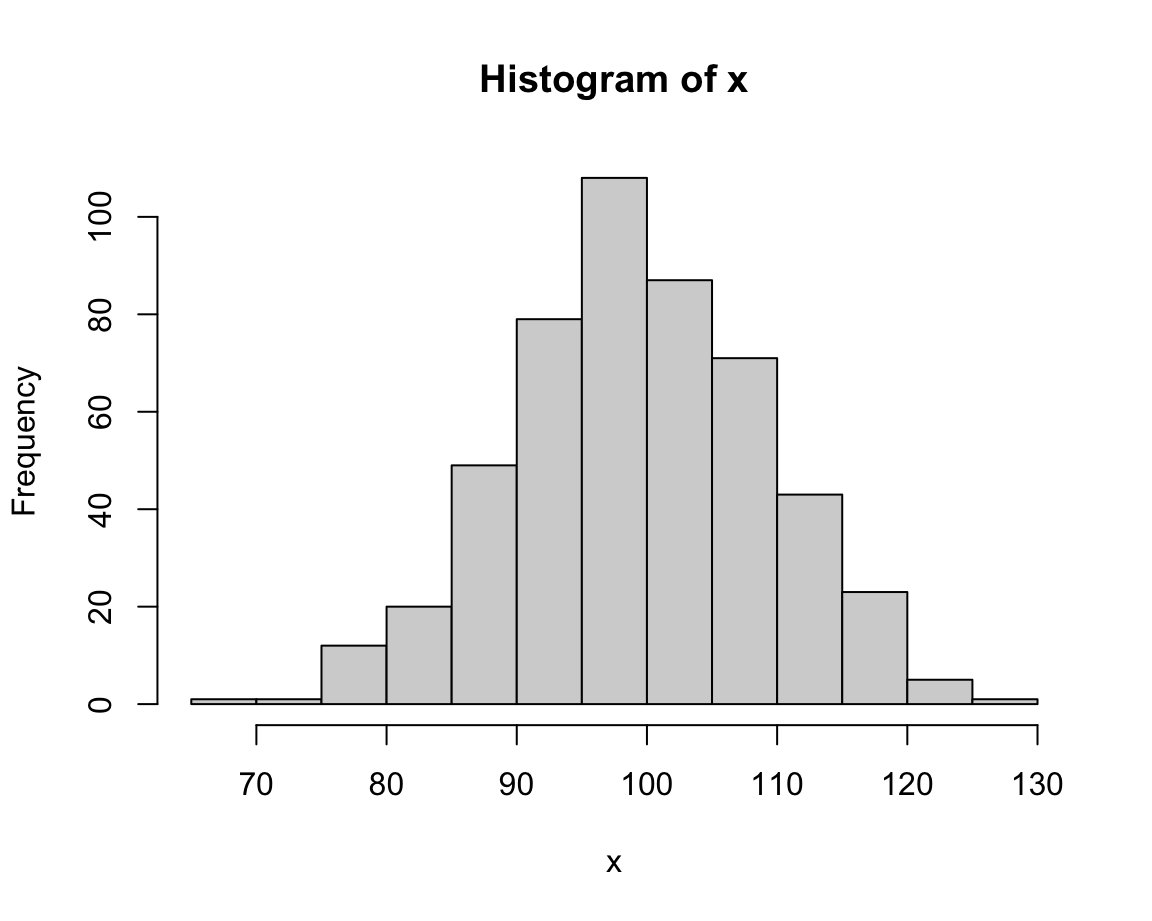This looks fairly straightforward, but due to the random nature of x the distribution of its values will vary every time we re-create the vector x.

Note that the hist() command added some automatic elements to our plot:

• a descriptive title (above the plot);
• an x- and a y-axes, with appropriate value ranges, axis ticks and labels;
• some geometric objects (here: vertical bars or rectangles) that represent the values of data.

Under the hood, the function even re-arranged the input vector and computed something: It categorized the values of x into bins of a specific width and counted the number of values falling into each bin (to show their frequency on the y-axis).

Whenever we are unhappy with the automatic defaults, we can adjust some parameters. In the case of histograms, an important parameter is the number of separate bins into which the data values are being categorized. This can be adjusted using the breaks argument:

# specifying breaks:
hist(x, breaks =  5)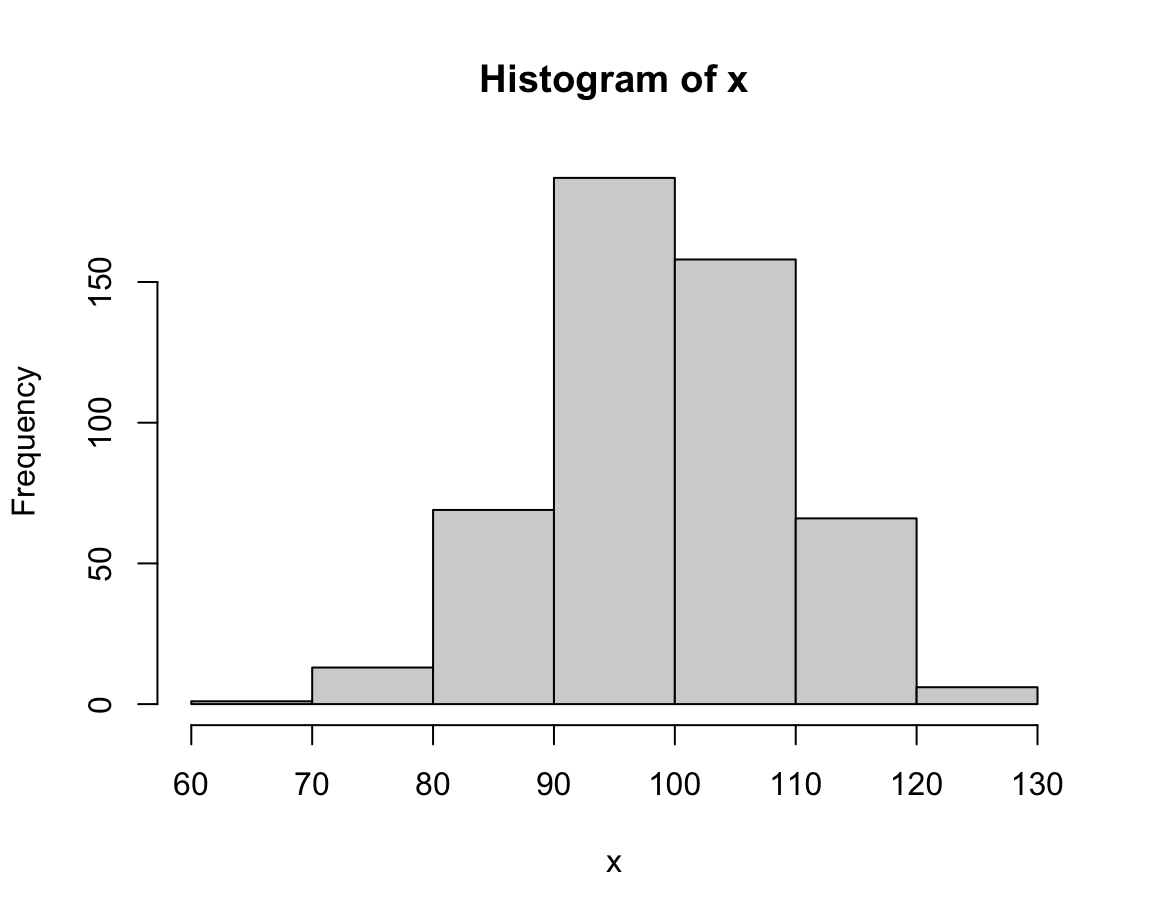hist(x, breaks = 25)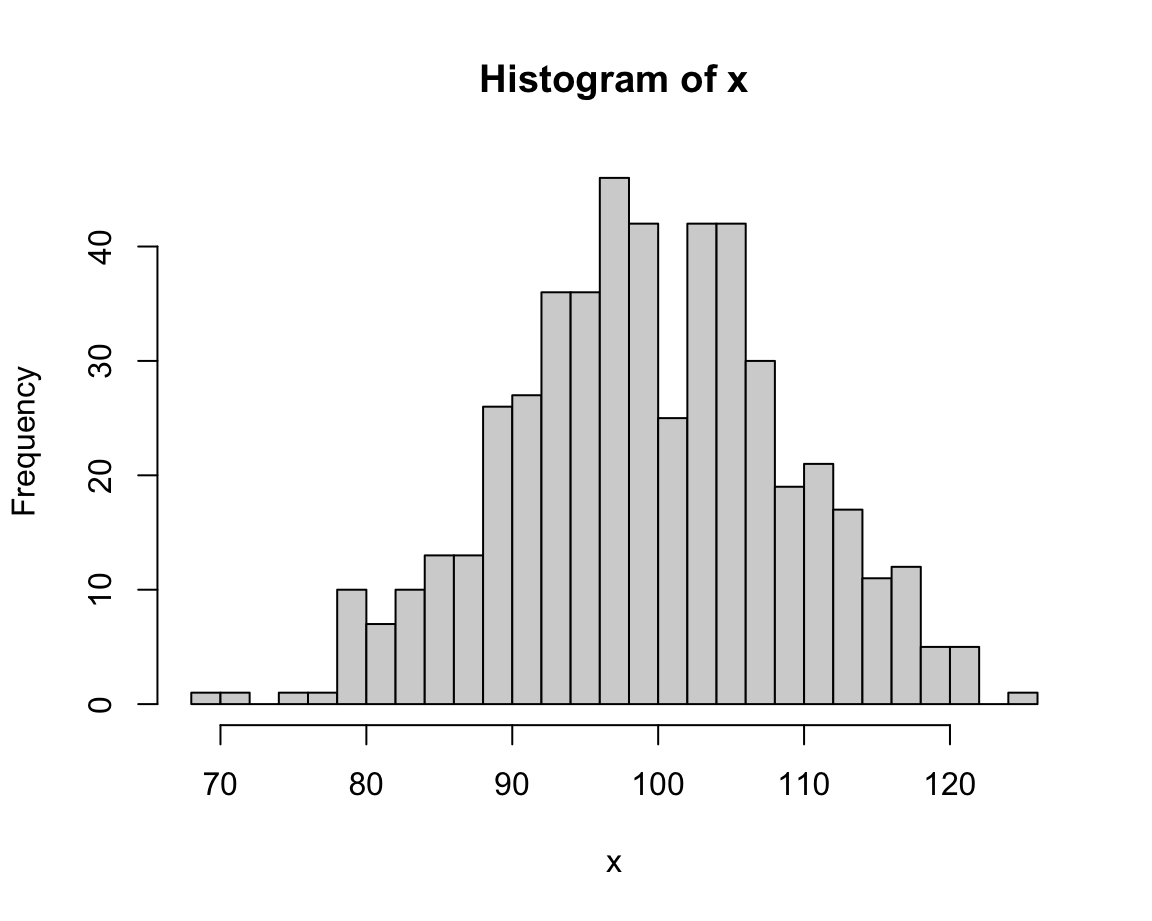Once we have settled on the basic parameters, we can adjust the labels and aesthetic aspects of a plot. A good plot should always contain informative titles, value ranges, and labels. In the following expression, we adjust the main title (using the main argument), the label of the x-Axis (using xlab argument), the main color of the bars and their border (using the col and border arguments):

# with aesthetics:
hist(x, breaks = 20,
main = "A basic histogram (showing the distribution of x)",
xlab = "Values of x",
col = "gold", border = "blue")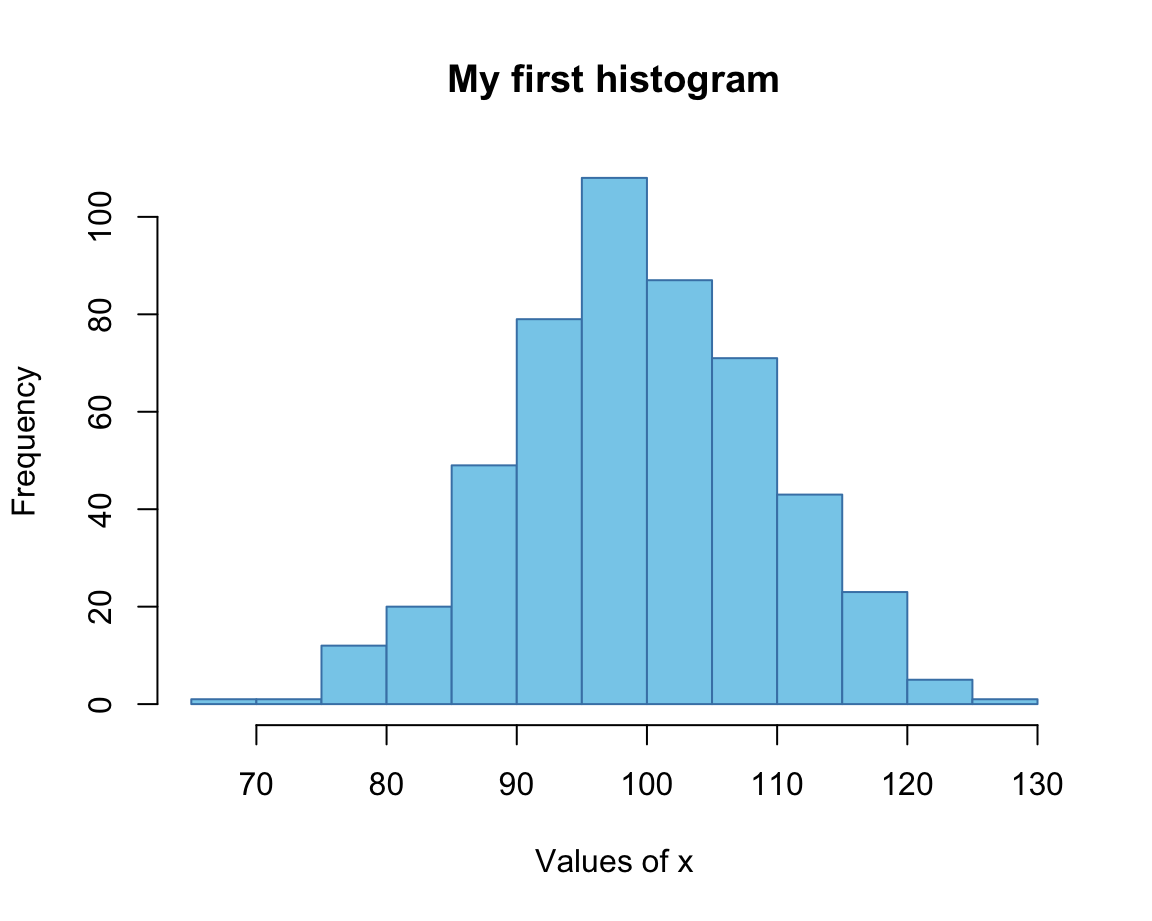Note that we did not adjust the range of values on the x- and y-axes. If we wanted to do this, we could have done so by providing the desired ranges (each as a vector with two numeric values) to the xlim and ylim arguments:

# with aesthetics:
hist(x, breaks = 20,
main = "A basic histogram (showing the distribution of x)",
xlab = "Values of x",
col = "gold", border = "red",
xlim = c(50, 150), ylim = c(0, 120))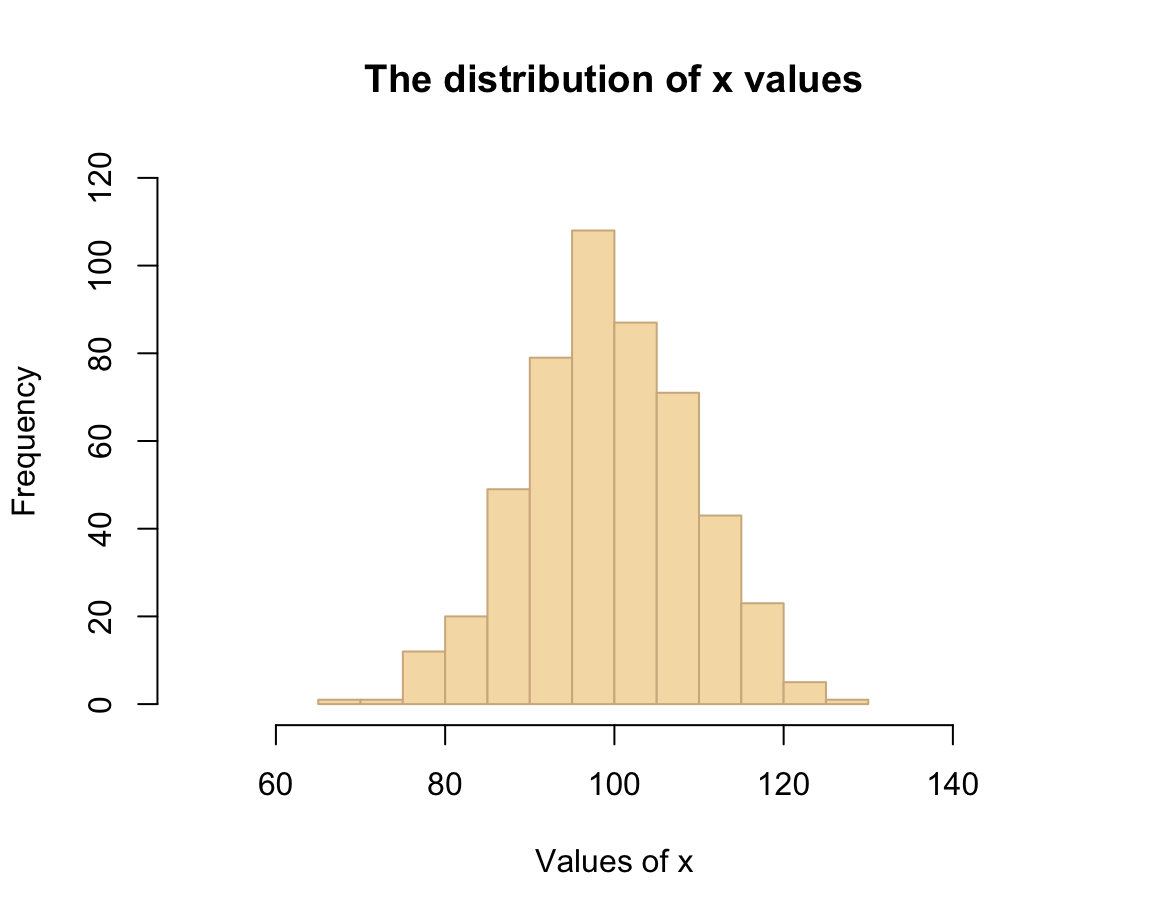#### Practice: Histogram of mpg data

Using the mpg data from the ggplot2 package, create a histogram that shows the distribution of values of the cty variable (showing a car’s fuel consumption, in miles per gallon (MPG), in the city).

Getting the data:

mpg <- ggplot2::mpg

Before starting to plot anything, we should always first inspect and try to understand our data:

# Print data table:
mpg  # a tibble with 234 cars, 11 variables

# Turn variable into a factor:
mpg$class <- factor(mpg$class)

# We are first interested in the vector
mpg$cty # Note: ?mpg # describes the data Solution: Here is how your histogram could look like: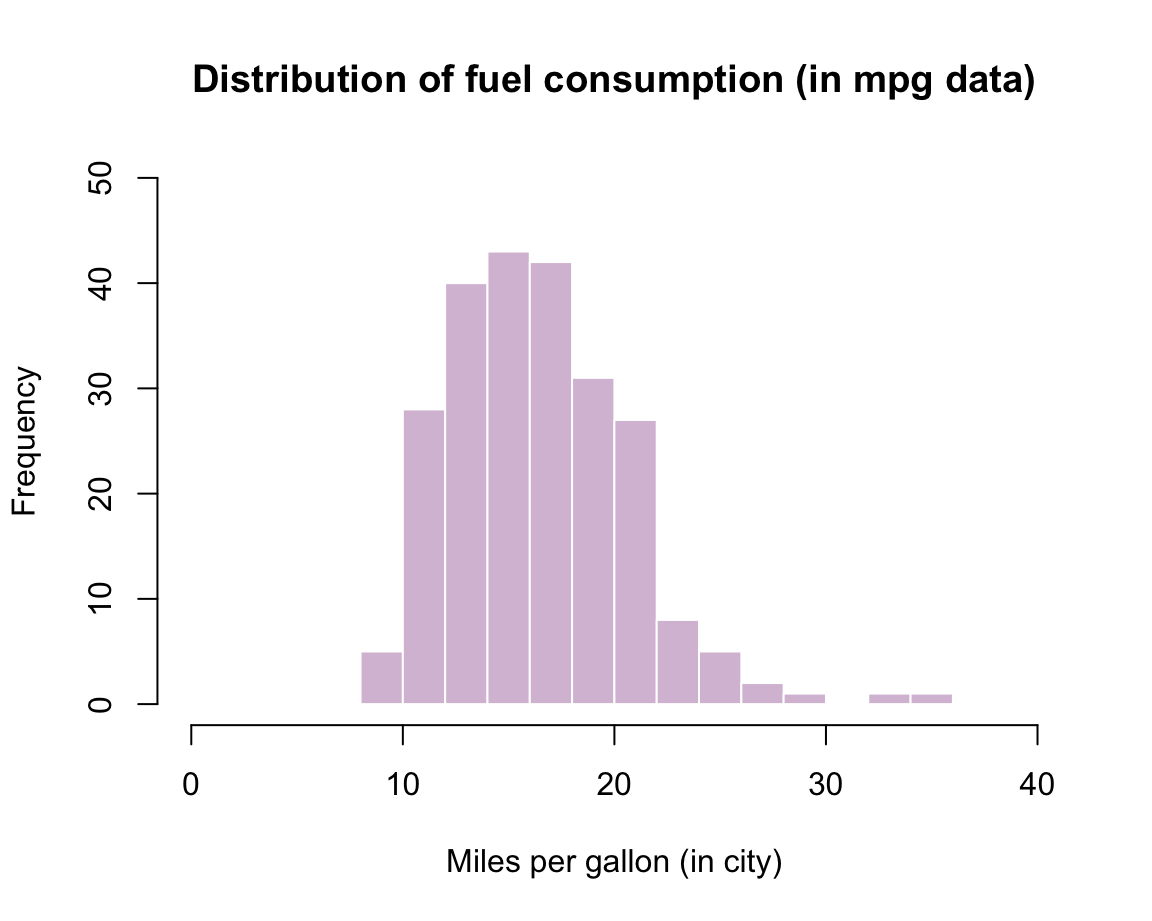### 4.3.2 Scatterplots The plot() function shows relationships between two variables x and y. Actually, plot() is a flexible plotting function in R: On the one hand, it allows defining new plots (e.g., create a new plotting canvas). On the other hand, calling the function with some data arguments aims to directly create a plot of them. In this section, we will call it with two vectors x and y to create a scatterplot (i.e., a plot of points). However, we will also see that plot() allows creating different plots of the same data, specifically: • a line plot; • a step function. We first create some data to plot. Here are two simple numeric vectors x and y (where y is defined as a function of x): # Data to plot: x <- -10:10 y <- x^2  When providing these vectors x and y to the x and y arguments of the plot() function, we obtain: # Minimal scatterplot: plot(x = x, y = y)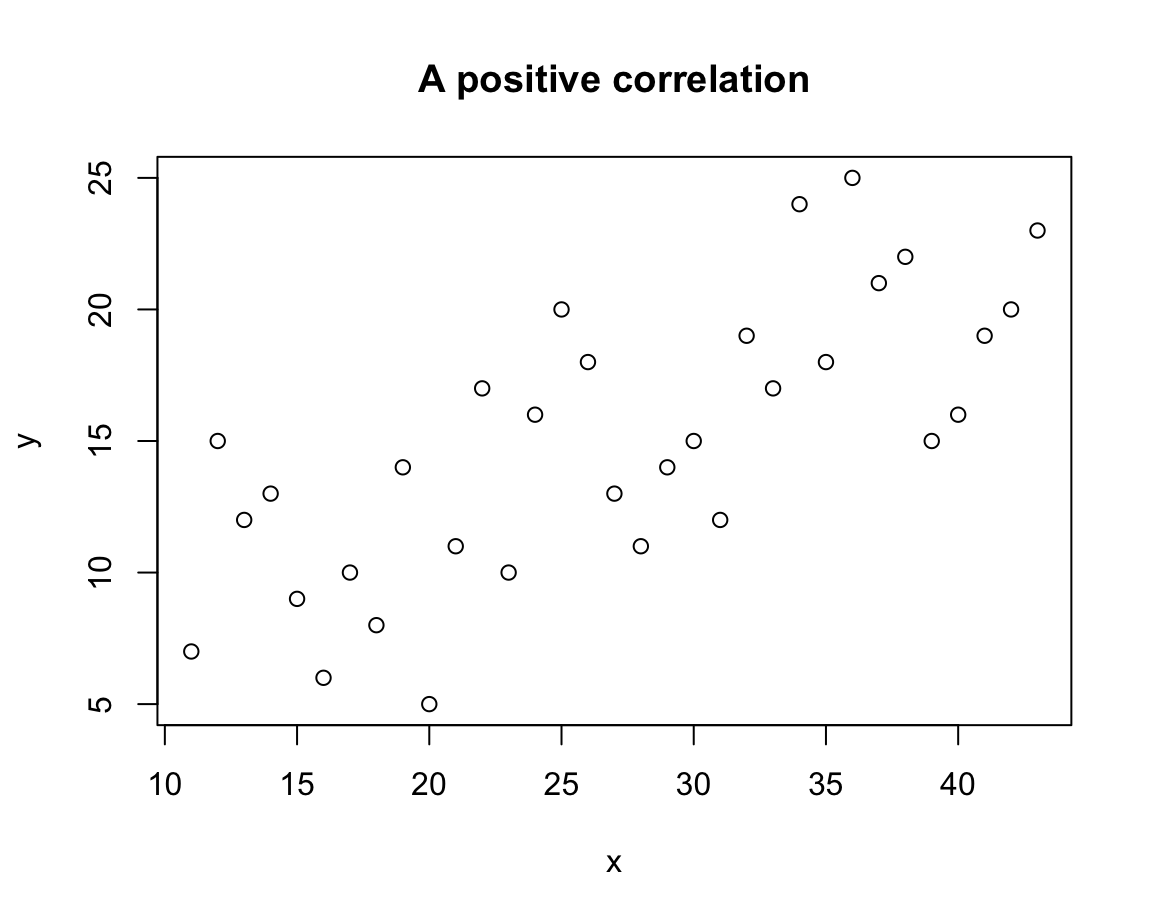Thus, the default plot chosen by plot() was a scatterplot (i.e., a plot of points). We can change the plot type by providing different values to the type argument: # Distinguish types: plot(x, y, type = "p") # points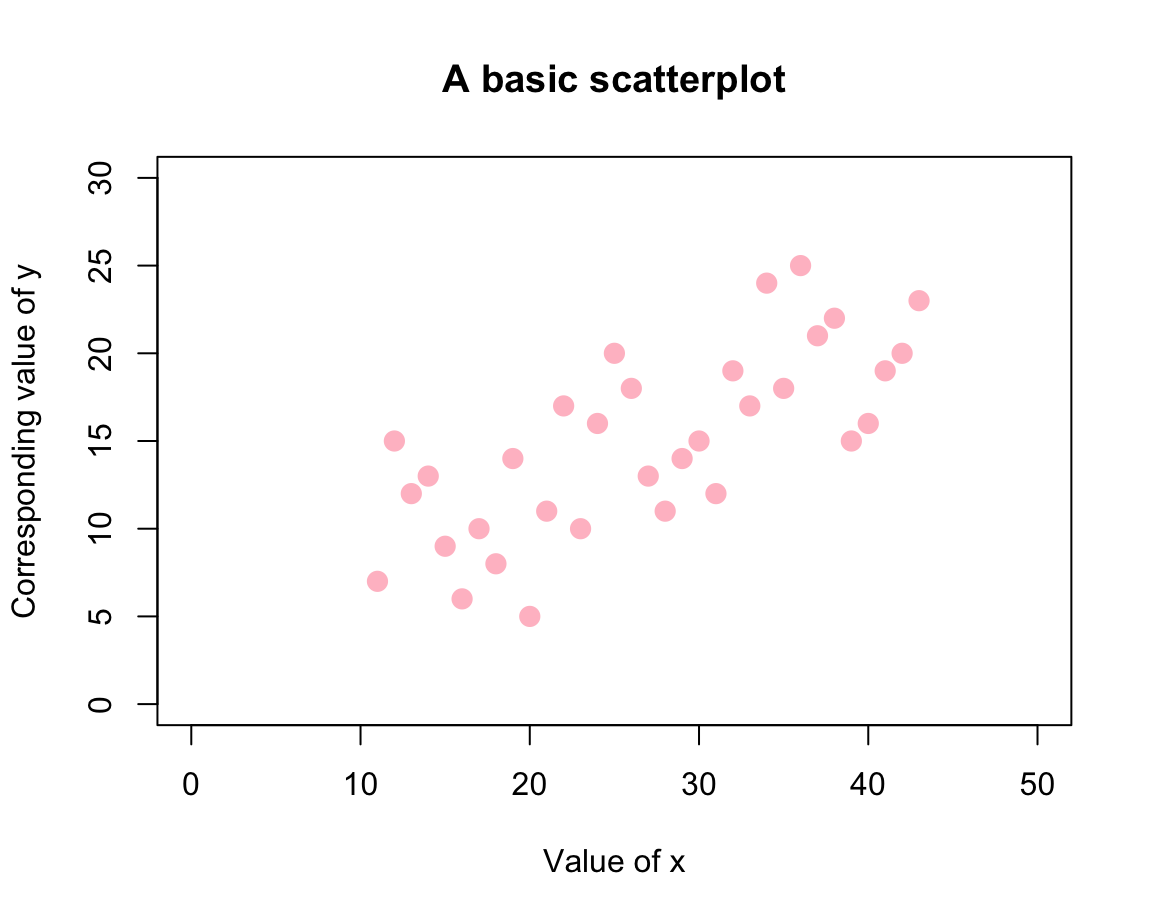plot(x, y, type = "l") # lines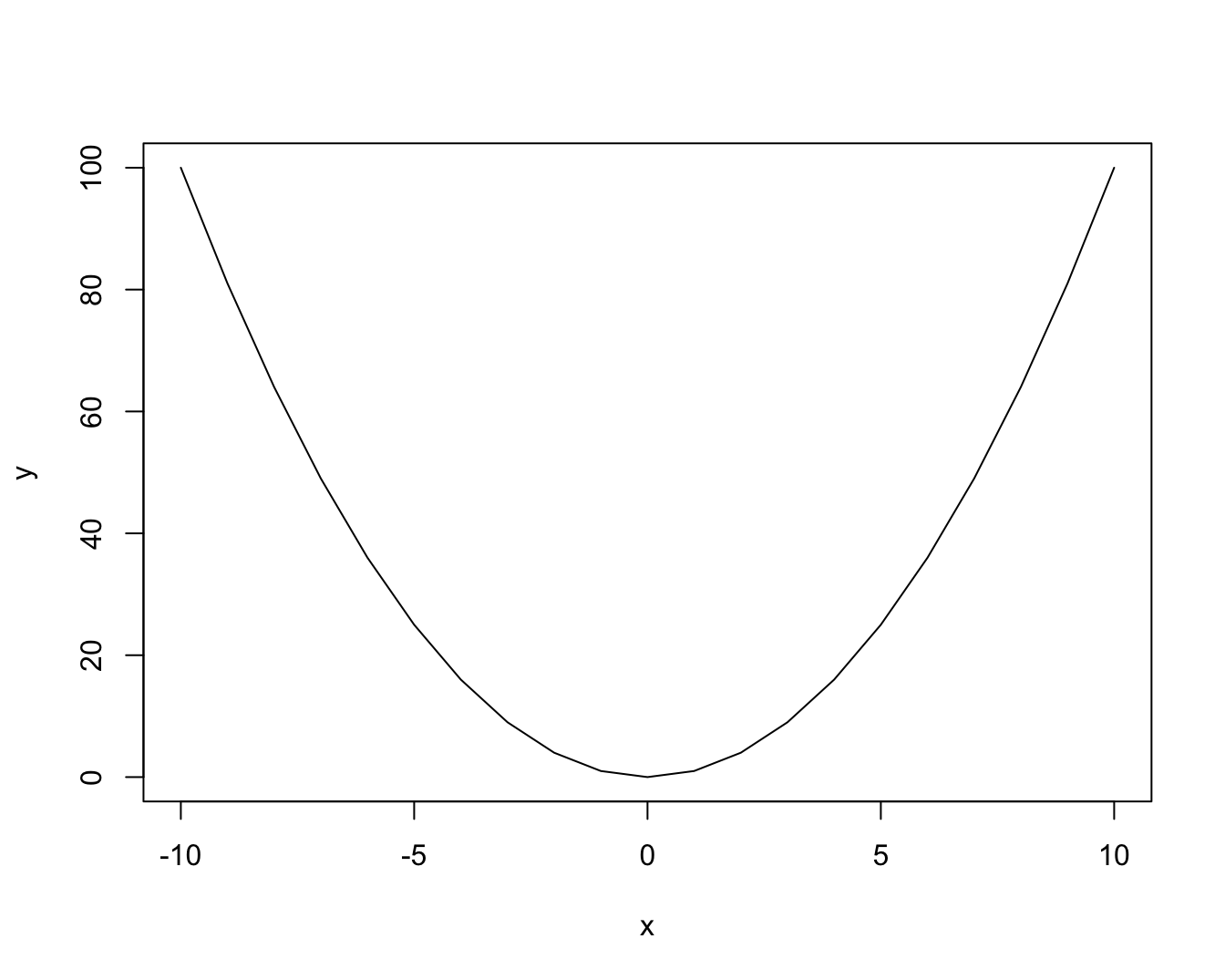plot(x, y, type = "b") # both points and lines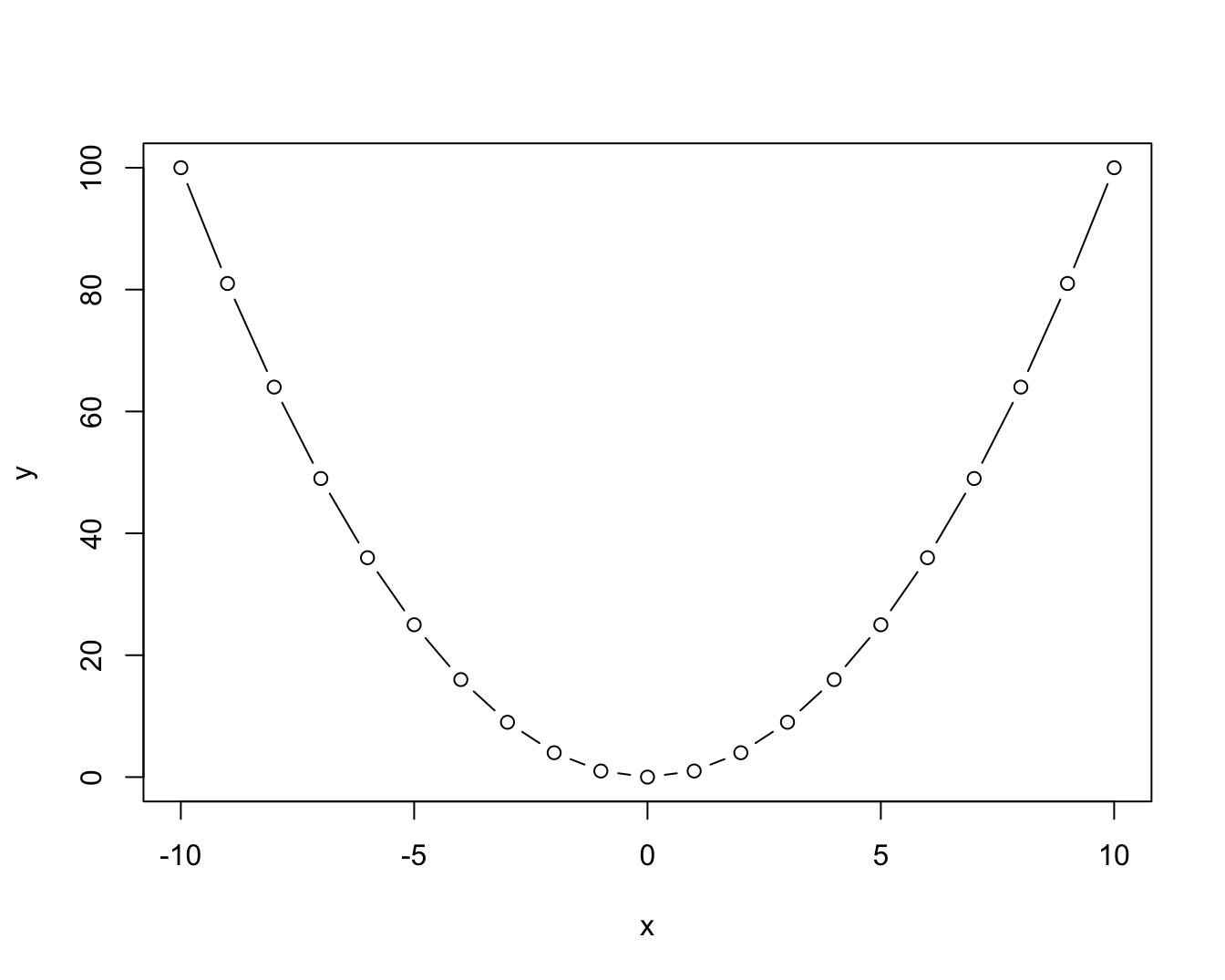plot(x, y, type = "o") # both overplotted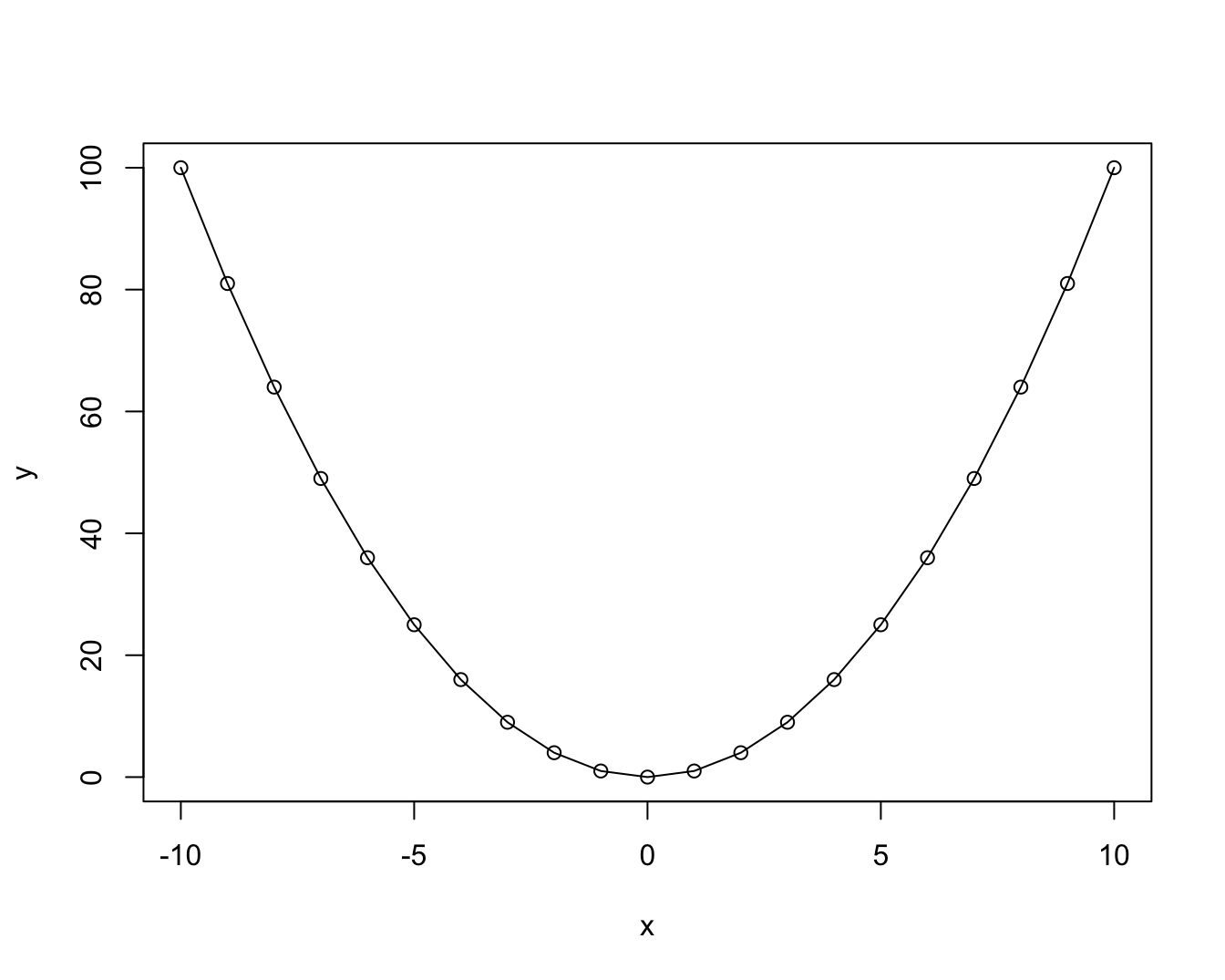plot(x, y, type = "h") # histogram or height density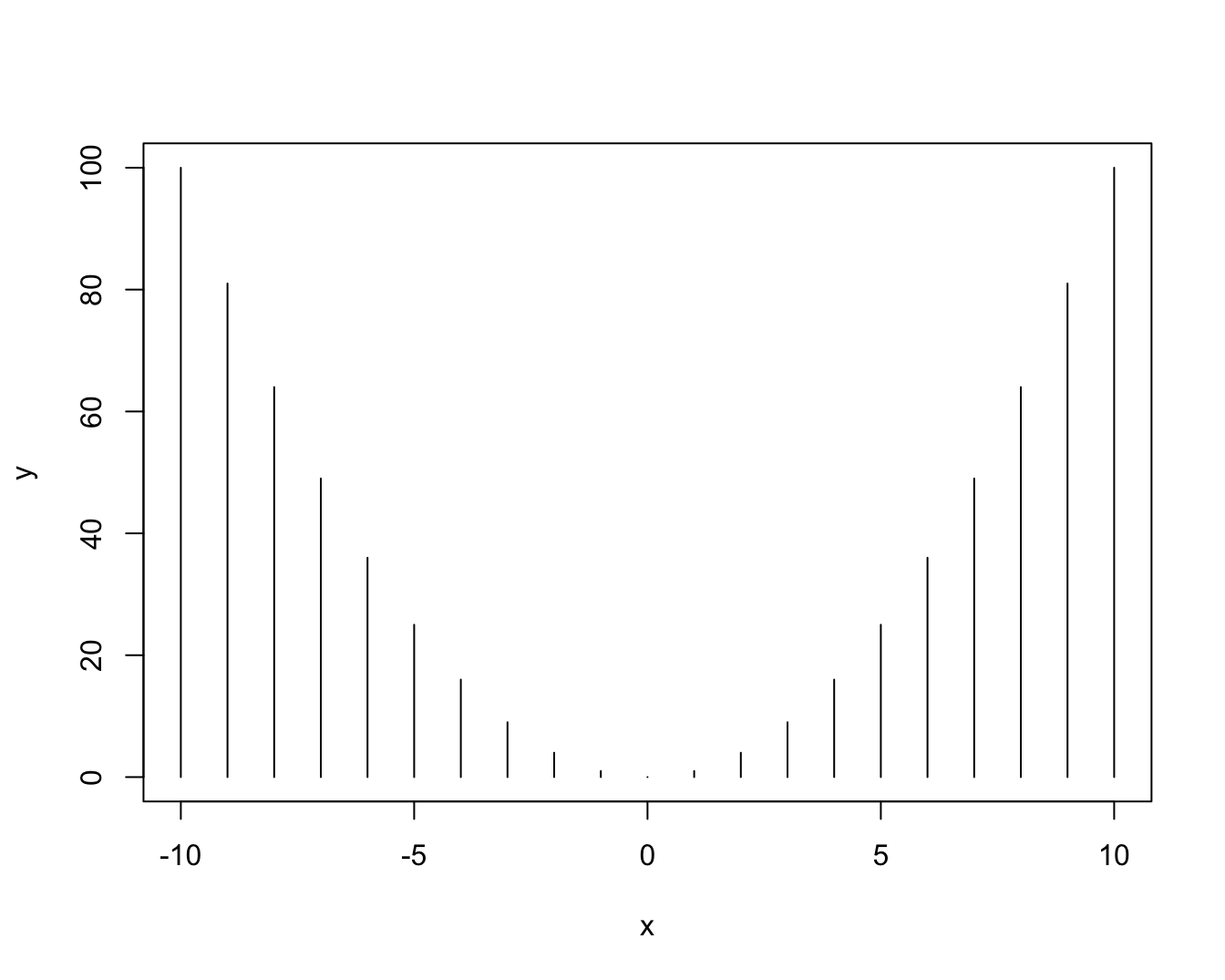plot(x, y, type = "s") # steps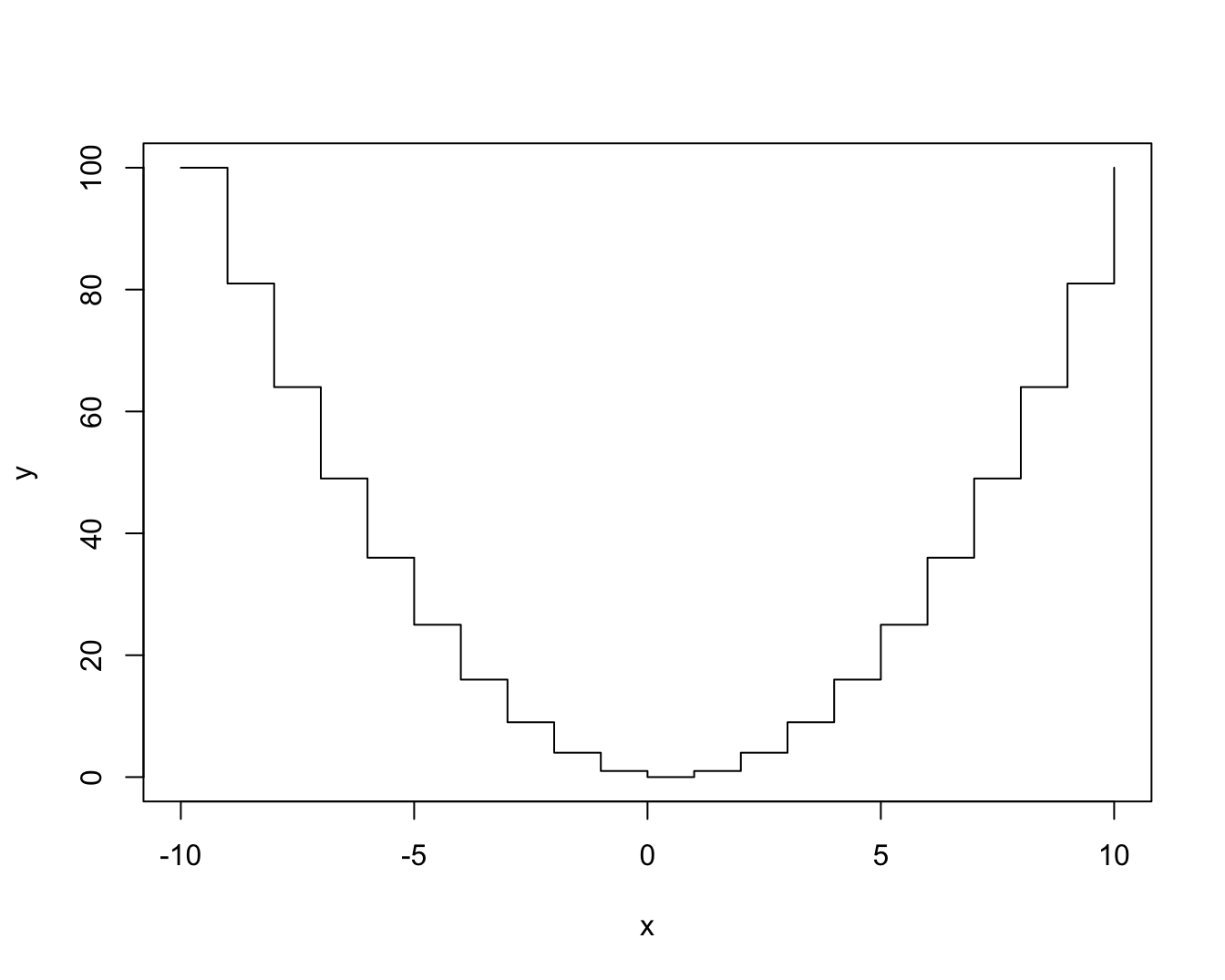See the documentation ?plot() for details on these types and additional arguments. For most datasets, only some of these types make sense. Actually, one of the most common uses of plot() uses the type n (for “no plotting”): plot(x, y, type = "n") # no plotting / nothing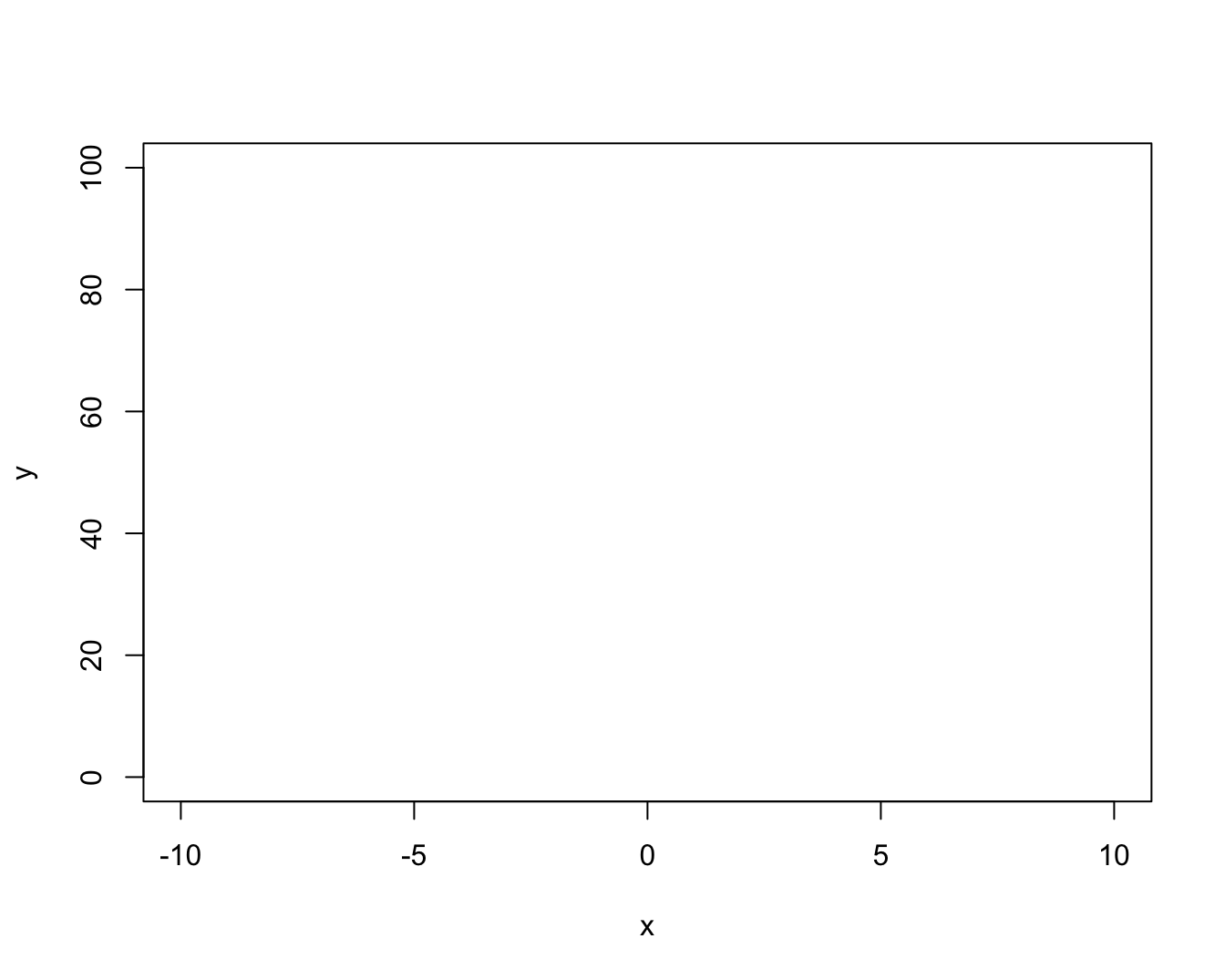As we see, this does not plot any data, but created an empty plot (with appropriate axis ranges). When creating more complex plots (below), we start like this and then add various custom objects to our plot. Once we have selected our plot, we can fiddle with its aesthetic properties and labels to make it both prettier and more informative: # Set aesthetic parameters and other options: plot(x, y, type = "b", lty = 2, pch = 16, lwd = 2, col = "red", cex = 1.5, main = "A basic plot (showing the relation between x and y)", xlab = "X label", ylab = "Y label", asp = 1/10 # aspect ratio (x/y) )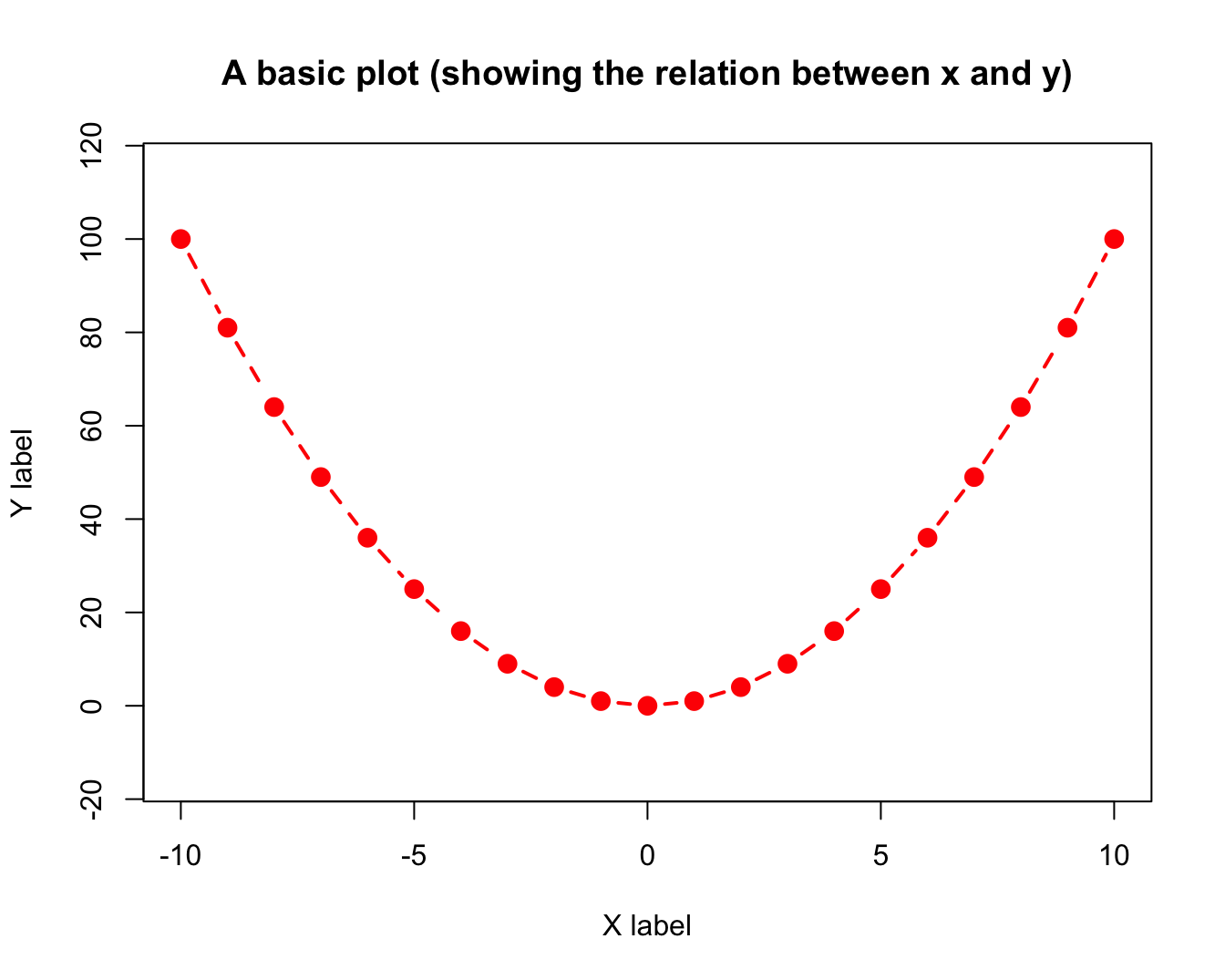#### Overplotting A common problem with scatterplots is overplotting (i.e., too many points at the same locations). For instance, suppose we wanted to plot the following data points: # Data to plot: x <- runif(250, min = 0, max = 10) y <- runif(250, min = 0, max = 10) Here is how basic scatterplot (with filled and fairly large points) would look like: # Basic scatterplot: plot(x, y, type = "p", pch = 20, cex = 4, # filled circles with increased size main = "An example of overplotting")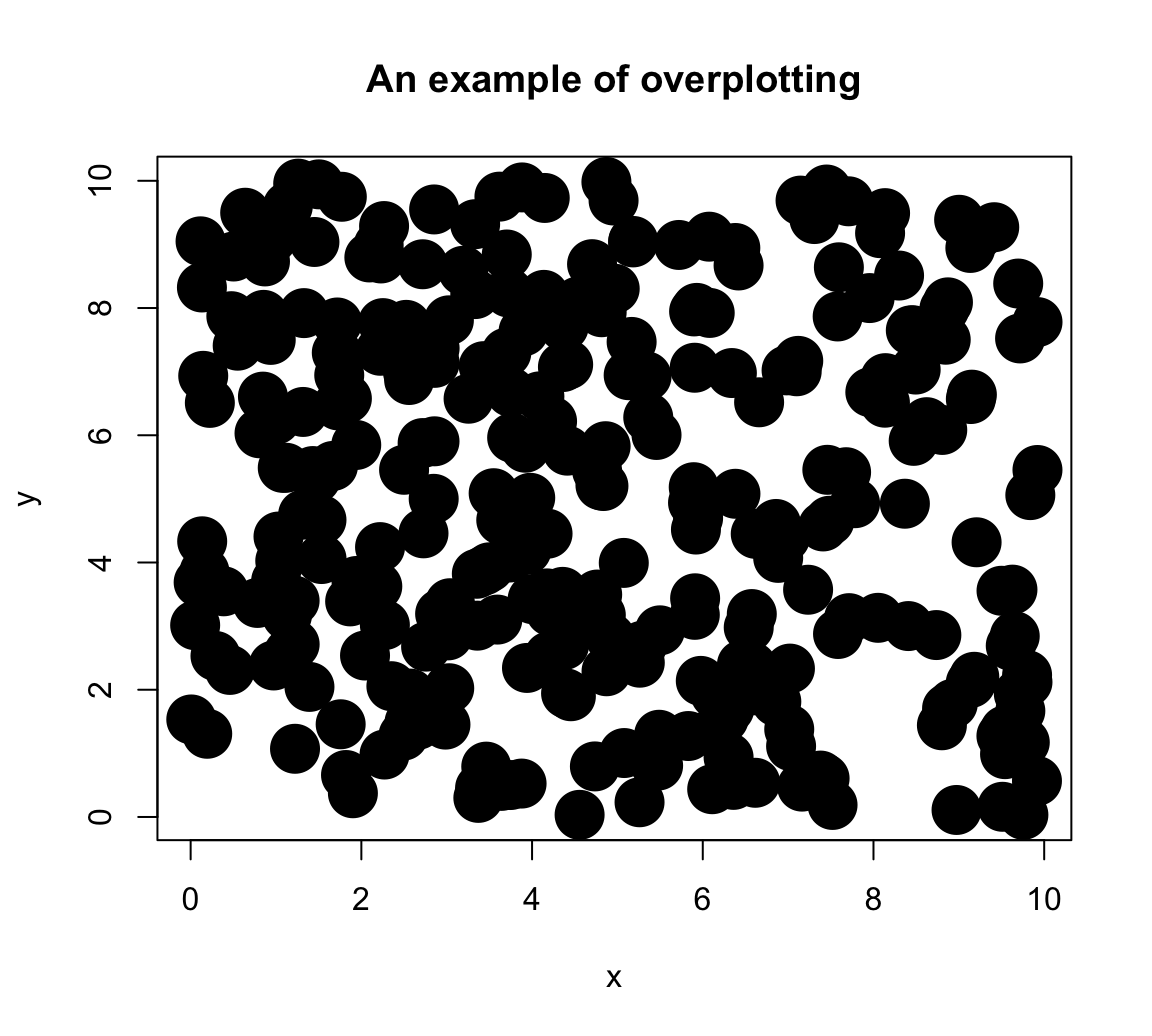Note: In case you’re wondering what pch and cex mean: • Calling ?par() provides detailed information on a large variety of graphical parameters. • Calling par() shows your current system settings. One of several solutions to the overplotting problem lies in using transparent colors, that allow viewing overlaps of graphical objects. There are several ways of obtaining transparent colors. For instance, the following solution uses the unikn package to select some dark color (called Petrol) and then use the usecol() function to set it to 1/3 of its original opacity: library(unikn) my_col <- usecol(Petrol, alpha = 1/3) Providing my_col to the col argument of plot() yields: # Set aesthetic parameters and other options: plot(x, y, type = "p", pch = 20, cex = 4, col = my_col, main = "Addressing overplotting (by color transparency)")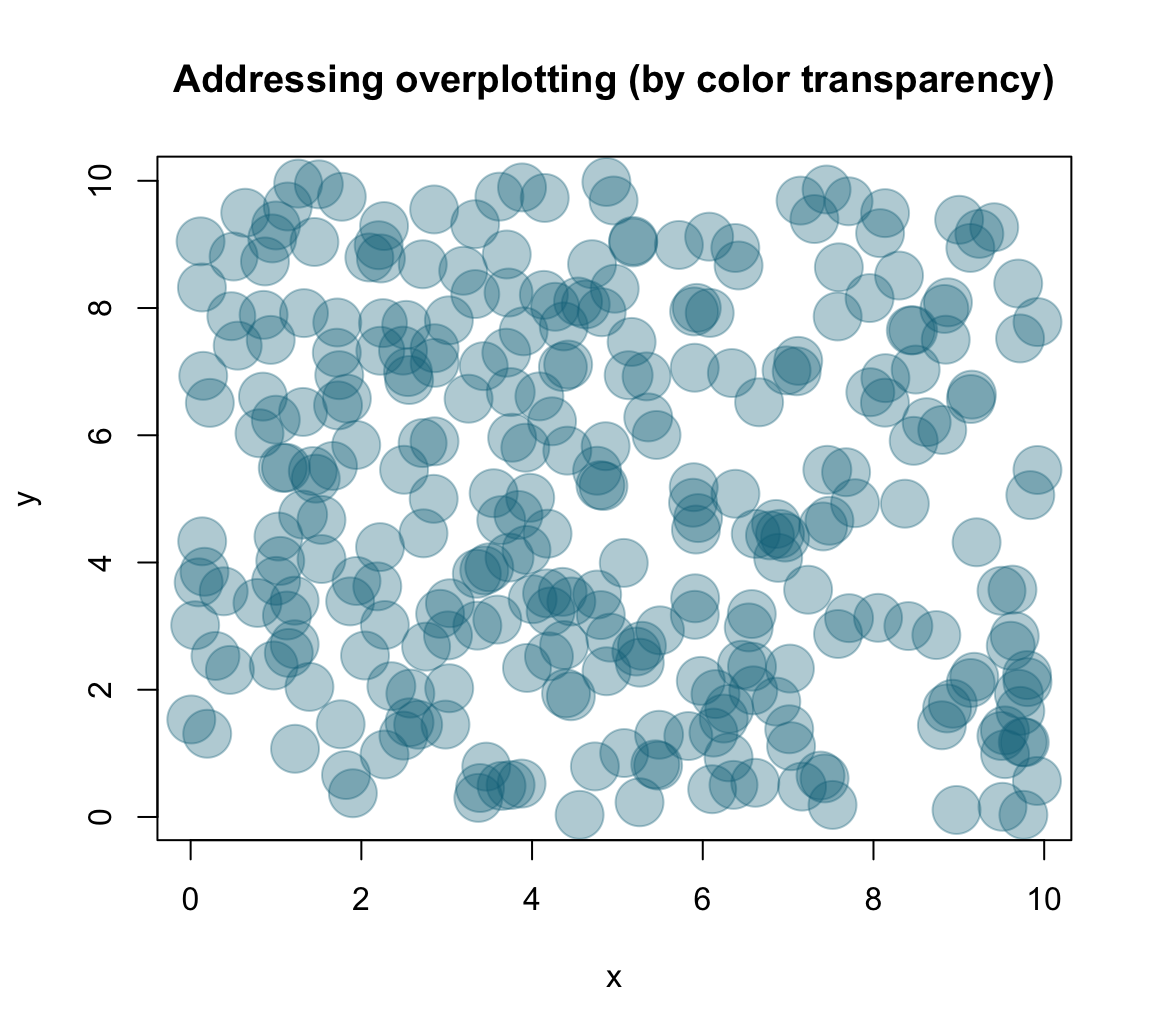Note that the following type variants of plot() may look pretty, but make very limited sense given this data: plot(x, y, type = "l", col = Seeblau) plot(x, y, type = "b", col = Pinky) plot(x, y, type = "h", col = Seegruen) plot(x, y, type = "s", col = Bordeaux)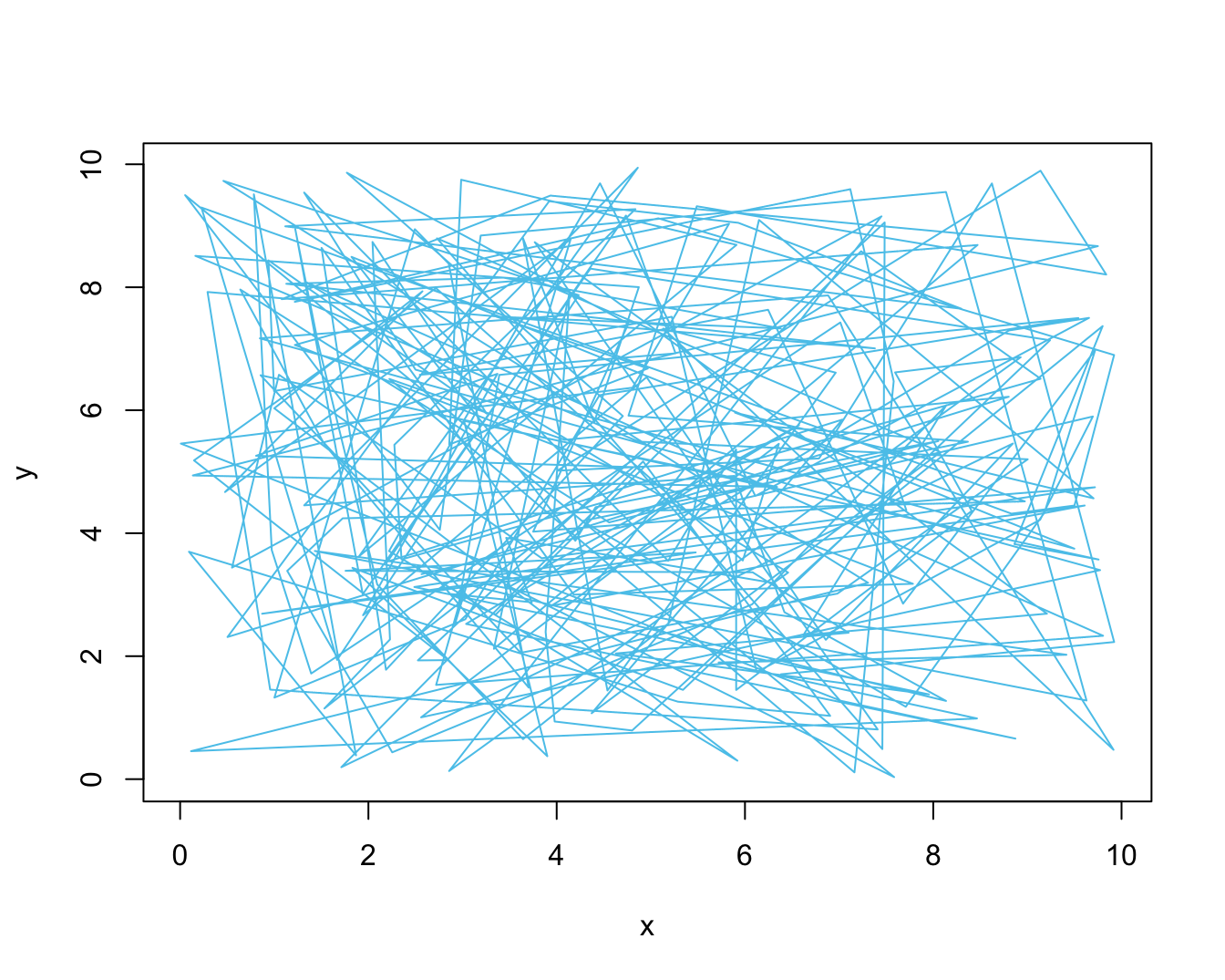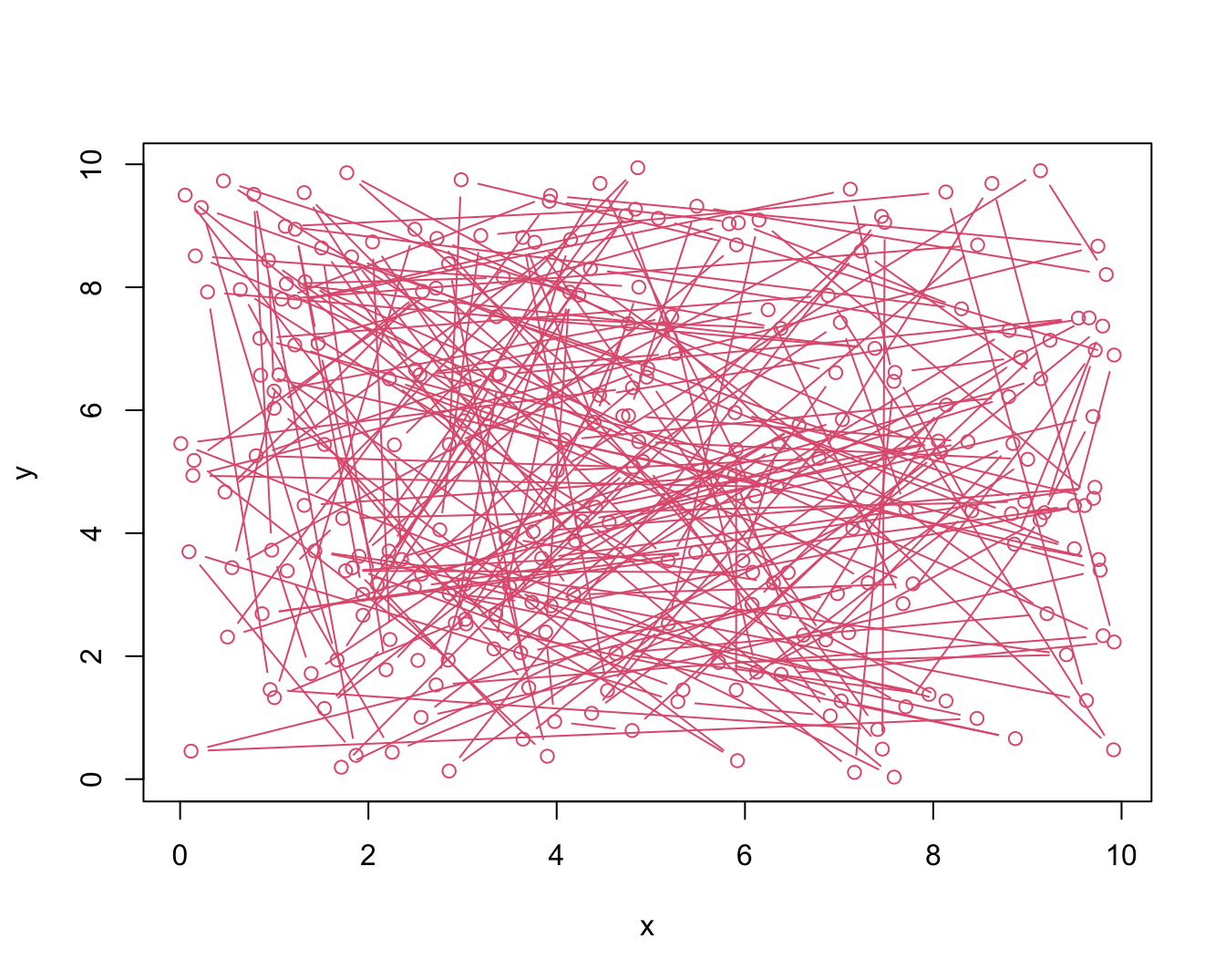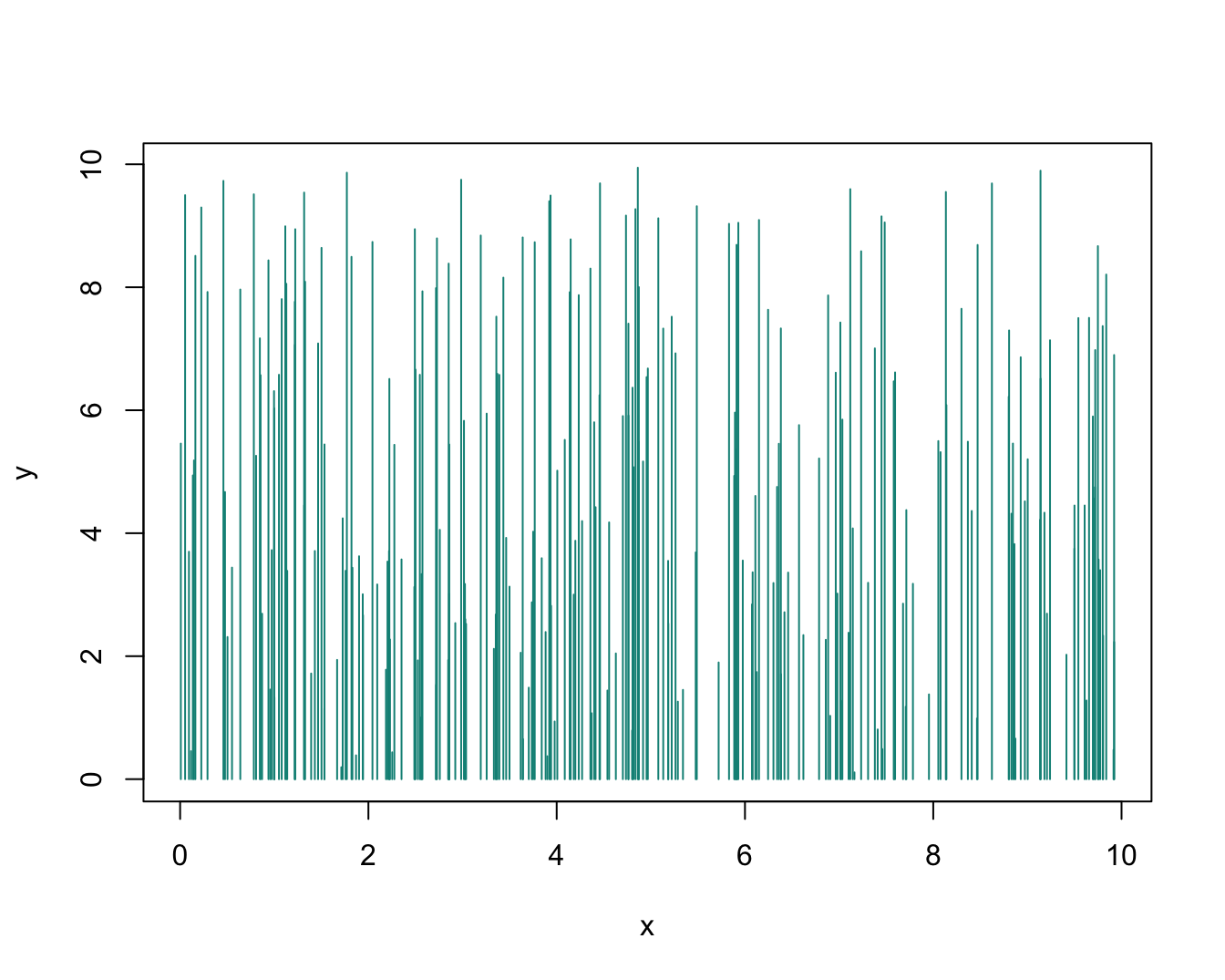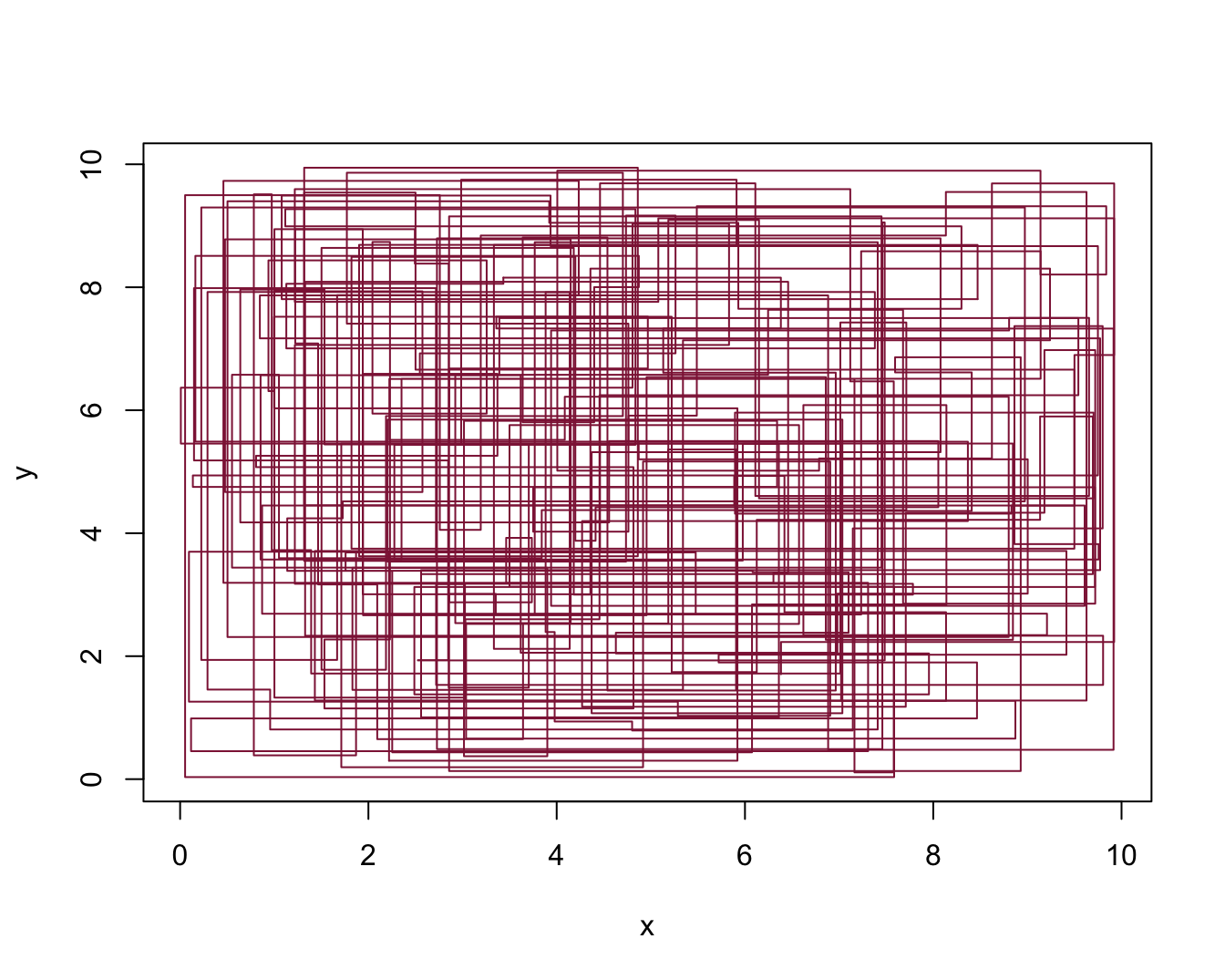Thus, which type of plot makes sense is primarily a function of the data that is to be shown.12 #### Practice: Scatterplots of mpg data Using variables from data: A typical scatterplot (using the mpg data from ggplot2): mpg <- ggplot2::mpg Create a scatterplot of this data that shows the relation between each car’s • x: engine displacement (i.e., variable displ of the mpg data), and • y: fuel consumption on highways (i.e., variable hwy of the mpg data). Can you avoid overplotting? # basic version: plot(x = mpg$displ, y = mpg$hwy)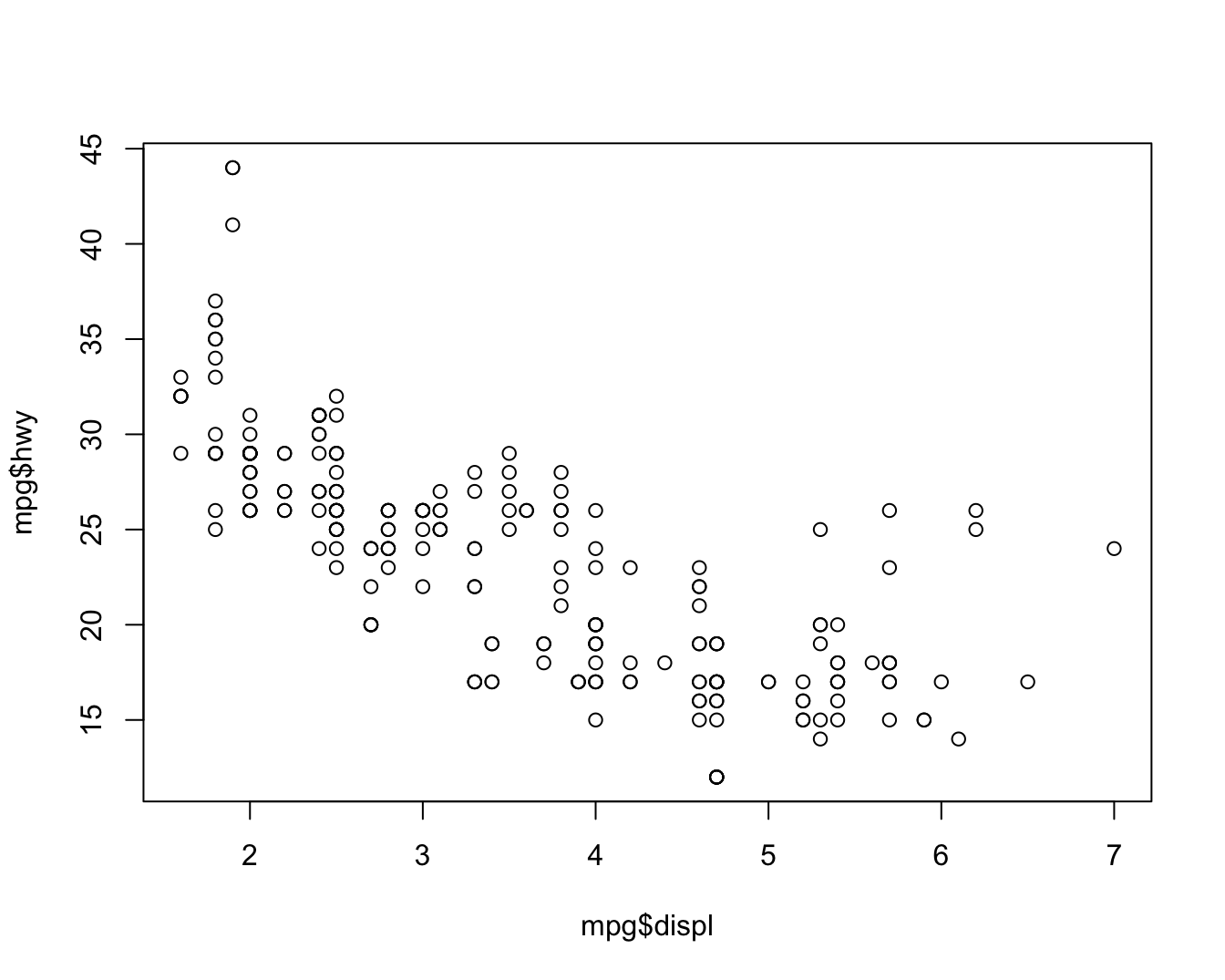# shorter version: with() with(mpg, plot(displ, hwy))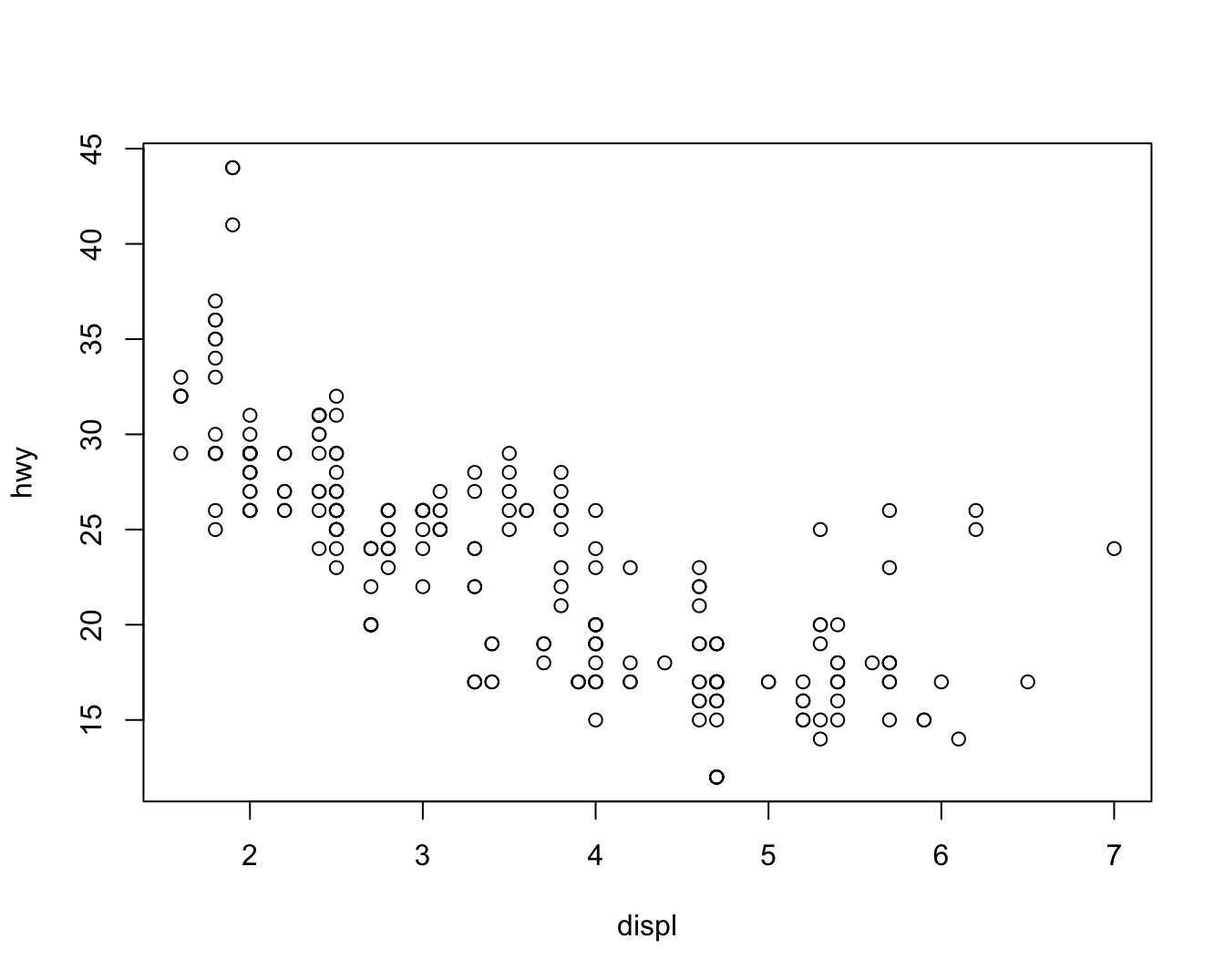# Define some color (from unikn, with transparency): my_col <- unikn::usecol(unikn::Bordeaux, alpha = 1/3) # With aesthetics (see ?par): plot(x = mpg$displ, y = mpg$hwy, type = "p", col = my_col, pch = 16, cex = 1.5, main = "A basic scatterplot (showing the relation between x and y)", xlab = "Displacement", ylab = "MPG on highway", asp = 1/10)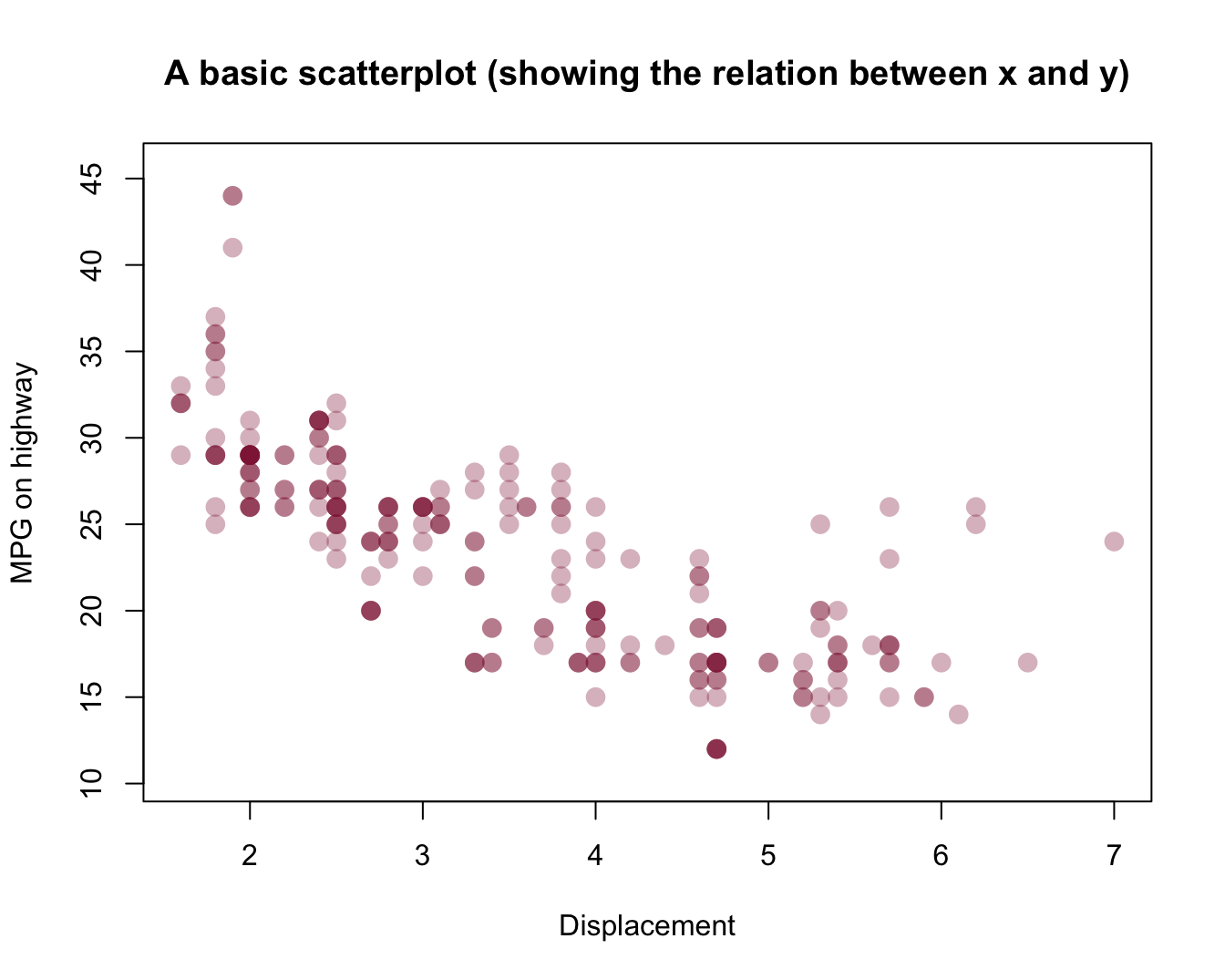Note: • plot dimensions (e.g., borders) using defaults • axes: ranges and values shown chosen automatically ### 4.3.3 Bar plots One of the most common, but also quite complicated types of plots are bar plots (aka. bar charts). The two main reasons why bar plots are complicated are: 1. the bars often represent processed data (e.g., counts, or the means, sums, or proportions of values). 2. the bars can be arranged in multiple ways (e.g., stacked vs. beside each other, grouped, etc.) When we have a named vector of data values that we want to plot, the barplot() command is pretty straightforward: # A vector as data: v <- c(1, 3, 2, 4, 2) # some values names(v) <- c(LETTERS[1:5]) # add names barplot(height = v, col = unikn::Seeblau)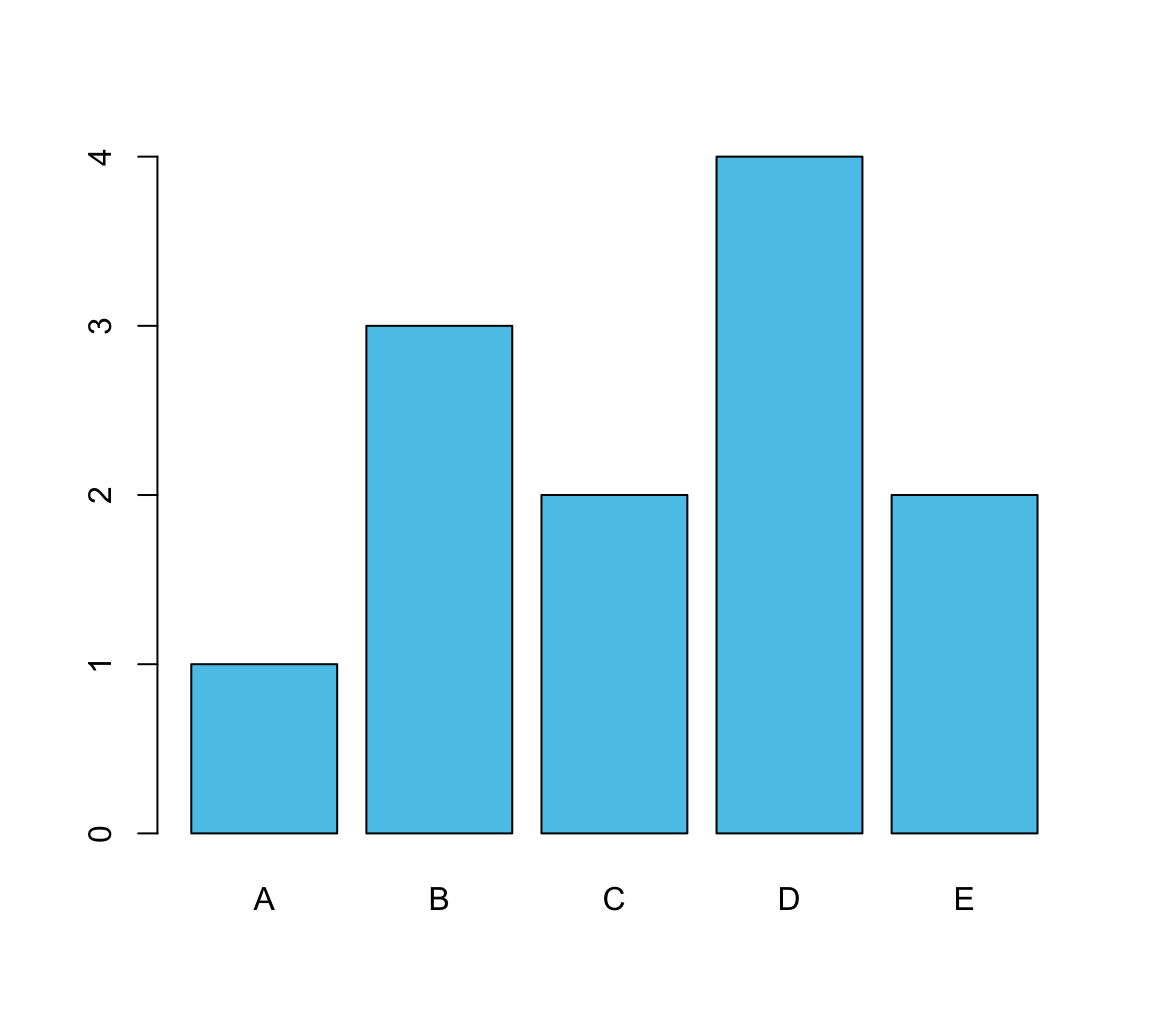In most cases, we have some more complicated data (e.g., a data frame or multiple vectors). To create a bar graph from data, we first create a table that contains the values we want to show. A simple example could use the mpg data from ggplot2: # From data: mpg <- ggplot2::mpg The following table() function creates a simple table of data by counting the number of observations (here: cars) for each level of the class variable: # Create a table (with frequency counts of cases): fc <- table(mpg$class)
# names(fc)
fc
#>
#>    2seater    compact    midsize    minivan     pickup subcompact        suv
#>          5         47         41         11         33         35         62

Providing this table as the height argument of barplot() creates a basic bar plot:

barplot(height = fc)  # basic version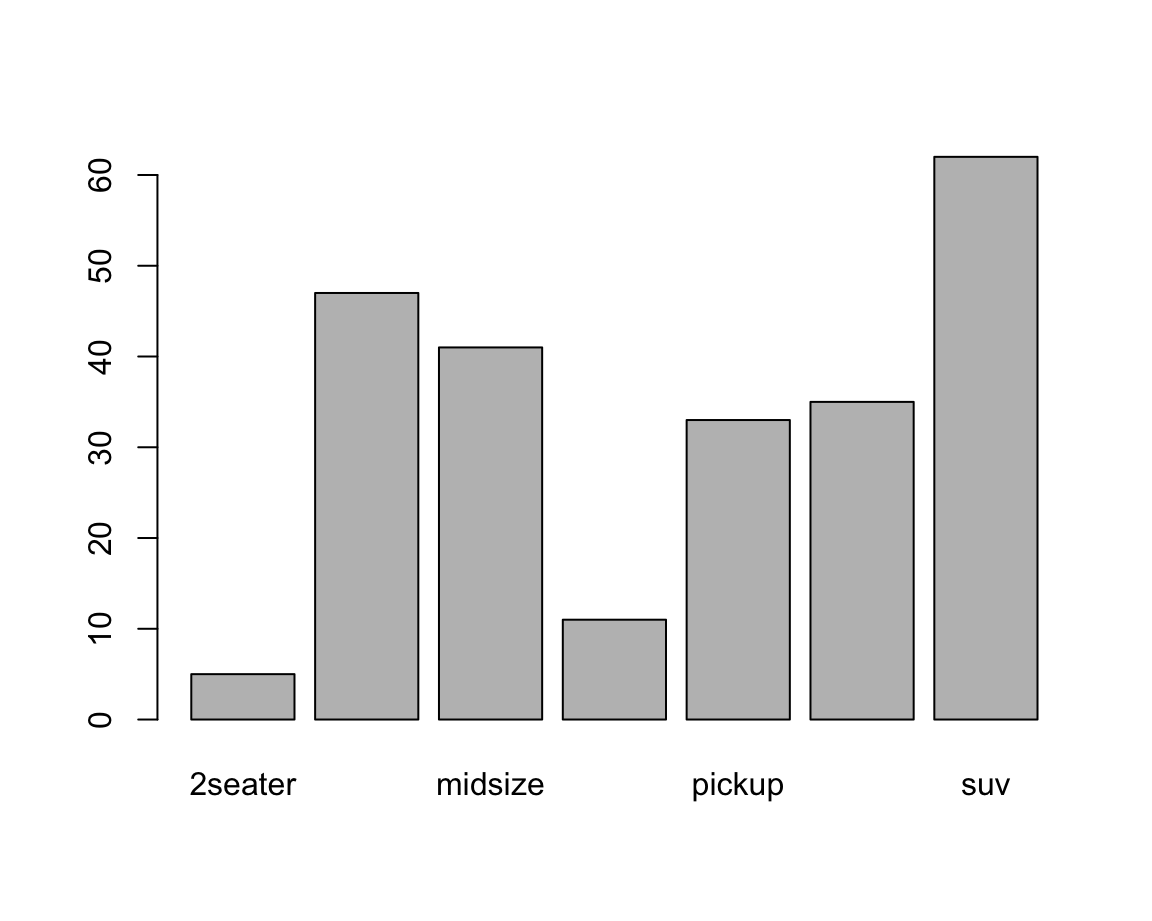Adding aesthetics and labels renders the plot more colorful and informative:

car_cols  <- unikn::usecol(pal_unikn_light)

barplot(height = fc,
main = "Counts of cars by class",
xlab = "Class of car",
las = 2,  # vertical labels
col = car_cols)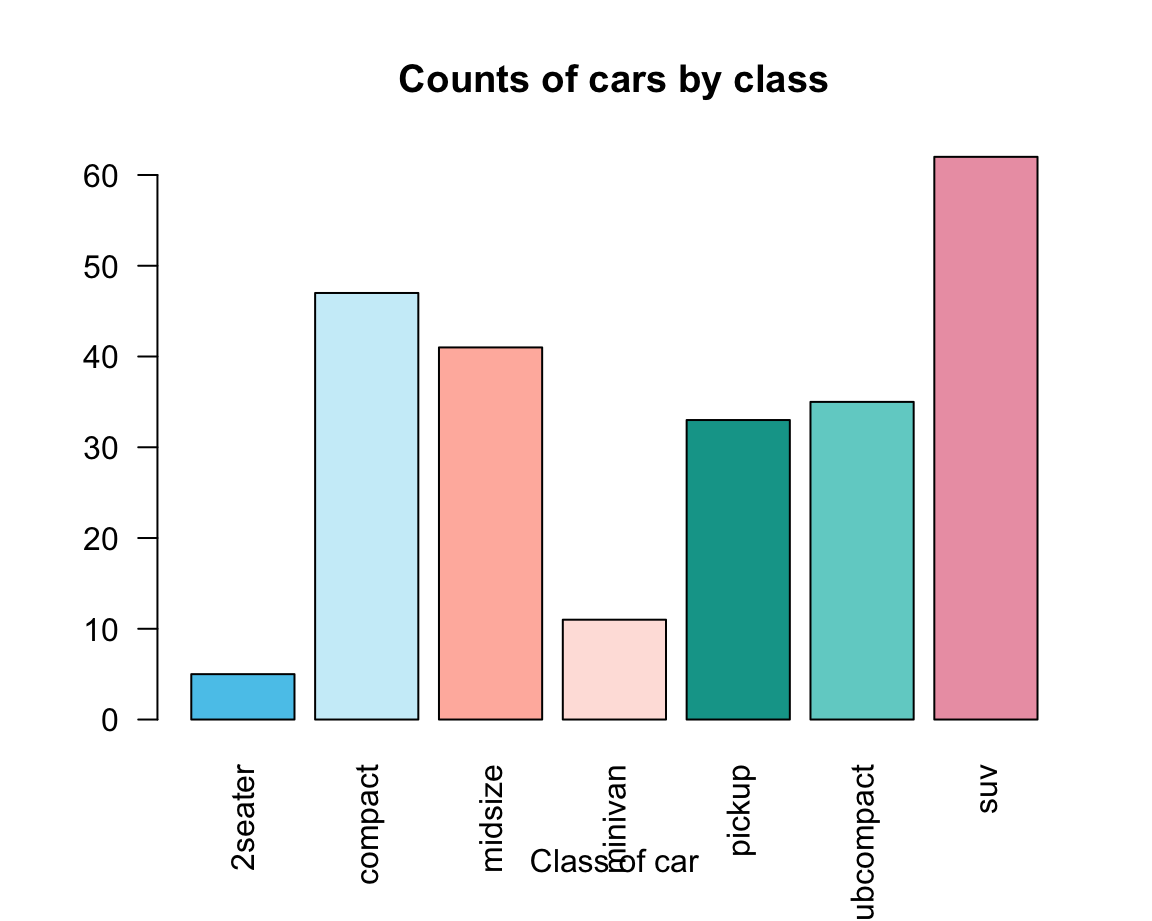An alternative way of creating a barplot() would use the data and formula arguments:

Using the UCBAdmissions data:

df <- as.data.frame(UCBAdmissions)

df_A <- df[df$Dept == "A", ] df_E <- df[df$Dept == "E", ]

# Select 2 colors:
my_cols <- c(Seeblau, Bordeaux)  # two colors

Create two bar plots:

# A:
barplot(data = df_A, Freq ~ Gender + Admit, beside = TRUE,
main = "Department A", col = my_cols, legend = TRUE)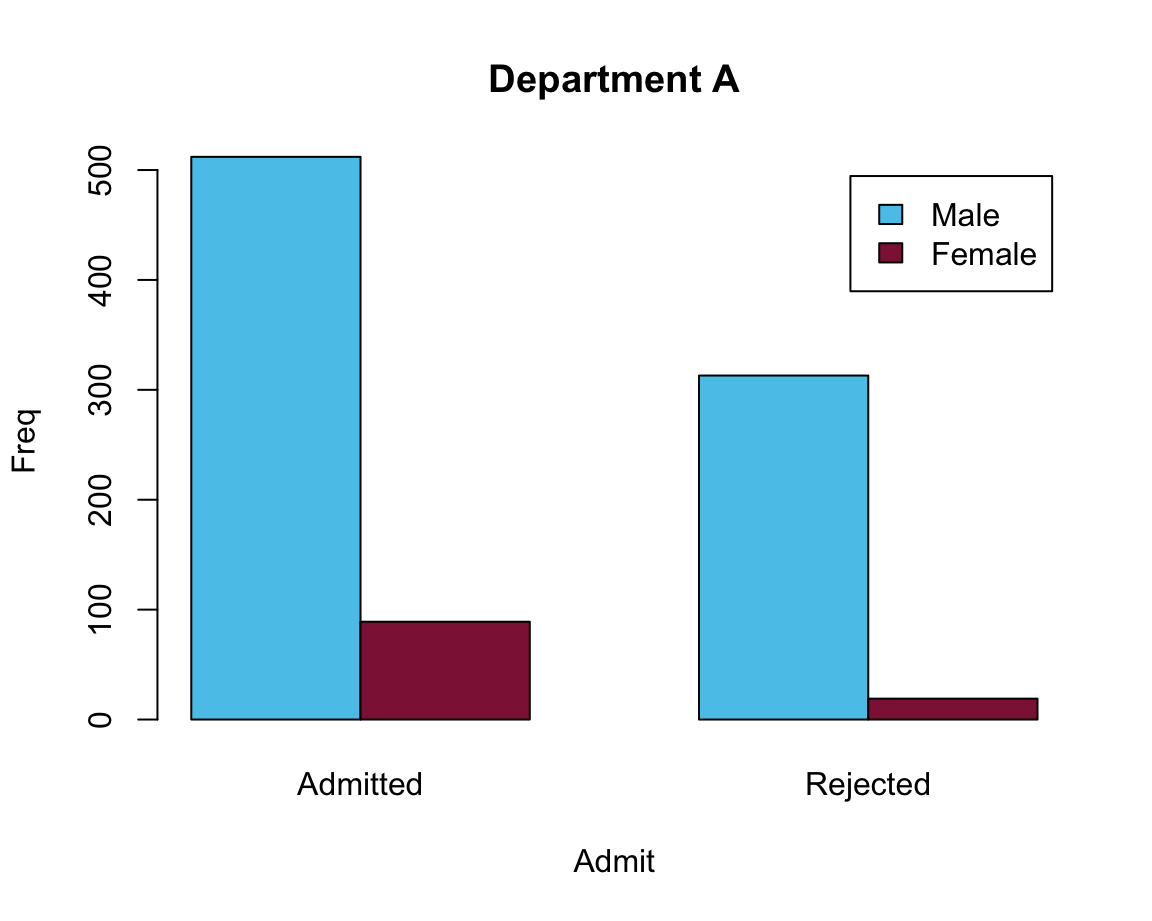# E:
barplot(data = df_E, Freq ~ Gender + Admit, beside = TRUE,
main = "Department E", col = my_cols, legend = TRUE)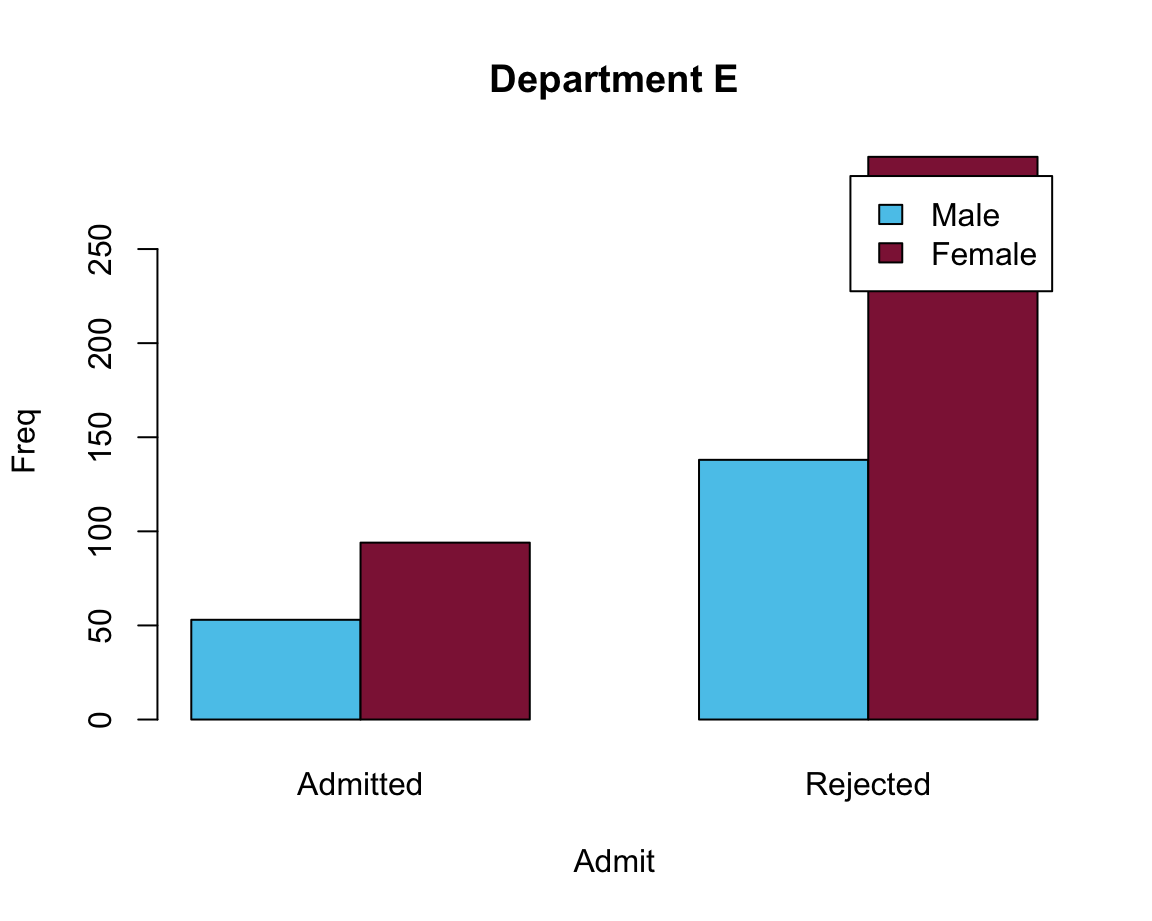Problem: Legend position overlaps with bars.

Two possible solutions:

# Moving legend position:
# Solution 1: specify args.legend (as a list)
barplot(data = df_E, Freq ~ Gender + Admit, beside = TRUE,
main = "Department E", col = my_cols,
legend = TRUE, args.legend = list(bty = "n", x = "topleft"))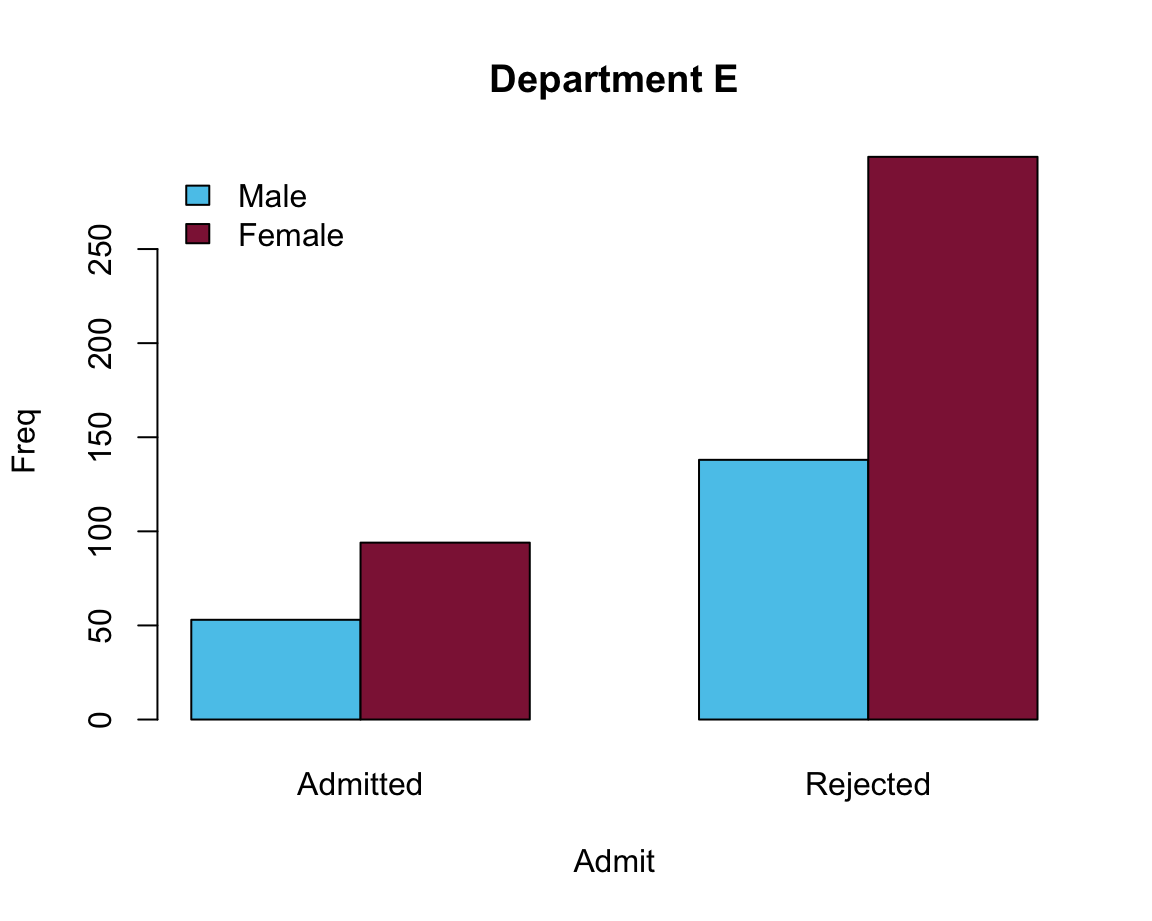# Solution 2: Adjust the size of the x-axis:
barplot(data = df_E, Freq ~ Gender + Admit, beside = TRUE,
main = "Department E", col = my_cols,
legend = TRUE, xlim = c(1, 8))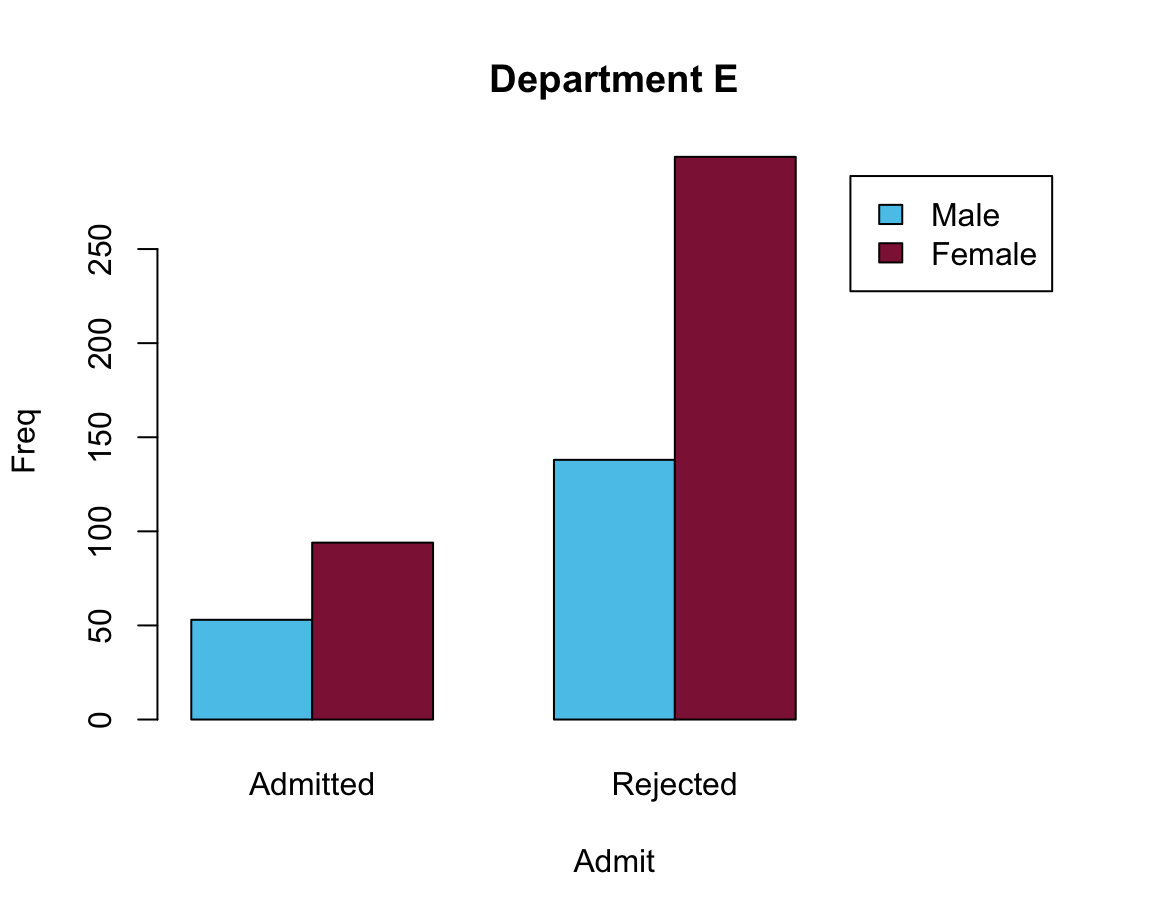#### Practice: Bar plots of election data

To practice our bar plot skills, we re-create some bar plots of election data shown here with base R commands:

1. with stacked bars (i.e., one bar per year);
1. with bars beside each other (i.e., three bars per year).

#### Data

Here is the election data from of a data table de (and don’t worry if the functions used to generate the data table are unknown at this point — we will cover them in the following chapters):

library(tidyverse)

# (a) Create data:
de_new <- data.frame(
party = c("CDU/CSU", "SPD", "Others"),
share_2013 = c((.341 + .074), .257, (1 - (.341 + .074) - .257)),
share_2017 = c((.268 + .062), .205, (1 - (.268 + .062) - .205))
)
de_new$party <- factor(de_new$party, levels = c("CDU/CSU", "SPD", "Others"))  # optional
# de_new

## Check that columns add to 100:
# sum(de_new$share_2013) # => 1 (qed) # sum(de_new$share_2017)  # => 1 (qed)

## (b) Converting de_new into a tidy tibble:
tb <- de_new %>%
gather(share_2013:share_2017, key = "election", value = "share") %>%
separate(col = "election", into = c("dummy", "year")) %>%
select(year, party, share)

# Choose colors:
my_col <- c("black", "firebrick", "gold")   # three specific colors
# my_col <- sample(x = colors(), size = 3)  # non-partisan alternative

# Show table:
knitr::kable(tb, caption = "Election data (2013 vs. 2017).")
Table 4.2: Election data (2013 vs. 2017).
year party share
2013 CDU/CSU 0.415
2013 SPD 0.257
2013 Others 0.328
2017 CDU/CSU 0.330
2017 SPD 0.205
2017 Others 0.465

#### Solution

Prior to plotting anything, we first select and define some colors that facilitate the interpretation of the plots:

my_cols <- c("black", "firebrick", "gold")  # define some colors

See colors() or demo(colors()) for color names available in R.

We can now solve the tasks using the barplot() function:

1. with stacked bars (i.e., one bar per year)

The following expressions plot a stacked bar plot (using the formula interface) and add colors and a legend:

# (a) Stacked bars:
barplot(data = tb, share ~ party + year)

barplot(data = tb, share ~ party + year,
col = my_cols, legend = TRUE)

However, a better solution could also re-size the x-axis (to position the legend at the right of the plot):

# Solution 1: limit x width to make space for legend to right:
barplot(data = tb, share ~ party + year,
col = my_cols, legend = TRUE, xlim = c(0, 4))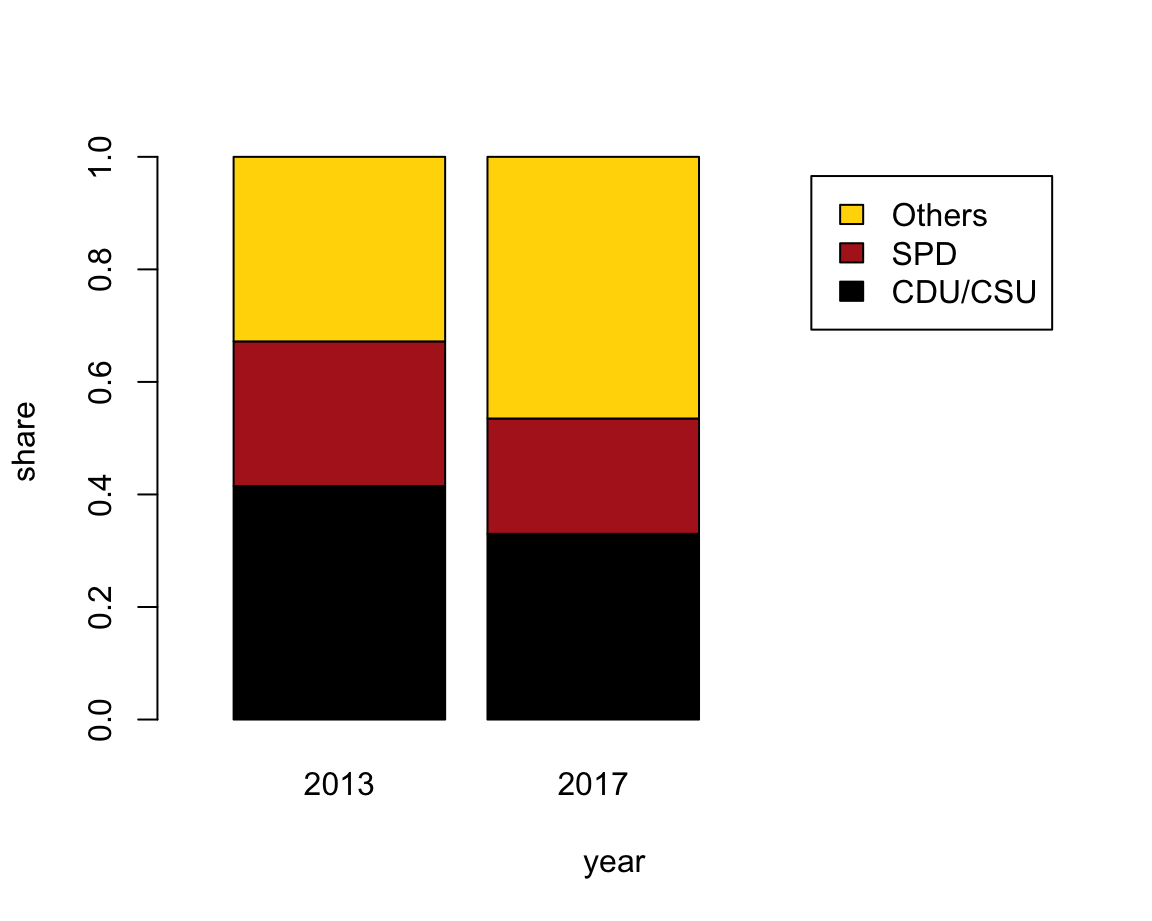1. with bars beside each other (i.e., three bars per year).

The following expression plots a bar plot with the bars beside each other and adds a legend:

# (b) Bars beside each other:
#     with legend (default position is "topleft"):
barplot(data = tb, share ~ party + year,
beside = TRUE, col = my_cols, legend = TRUE)

Again, a better solution would position the legend and re-size the limits of the y-axis:

# Solution 2: Adjust legend parameters (to move in center and no border):
barplot(data = tb, share ~ party + year,
beside = TRUE, col = my_cols,
legend = TRUE, args.legend = list(bty = "n", x = "top"),
ylim = c(0, .5))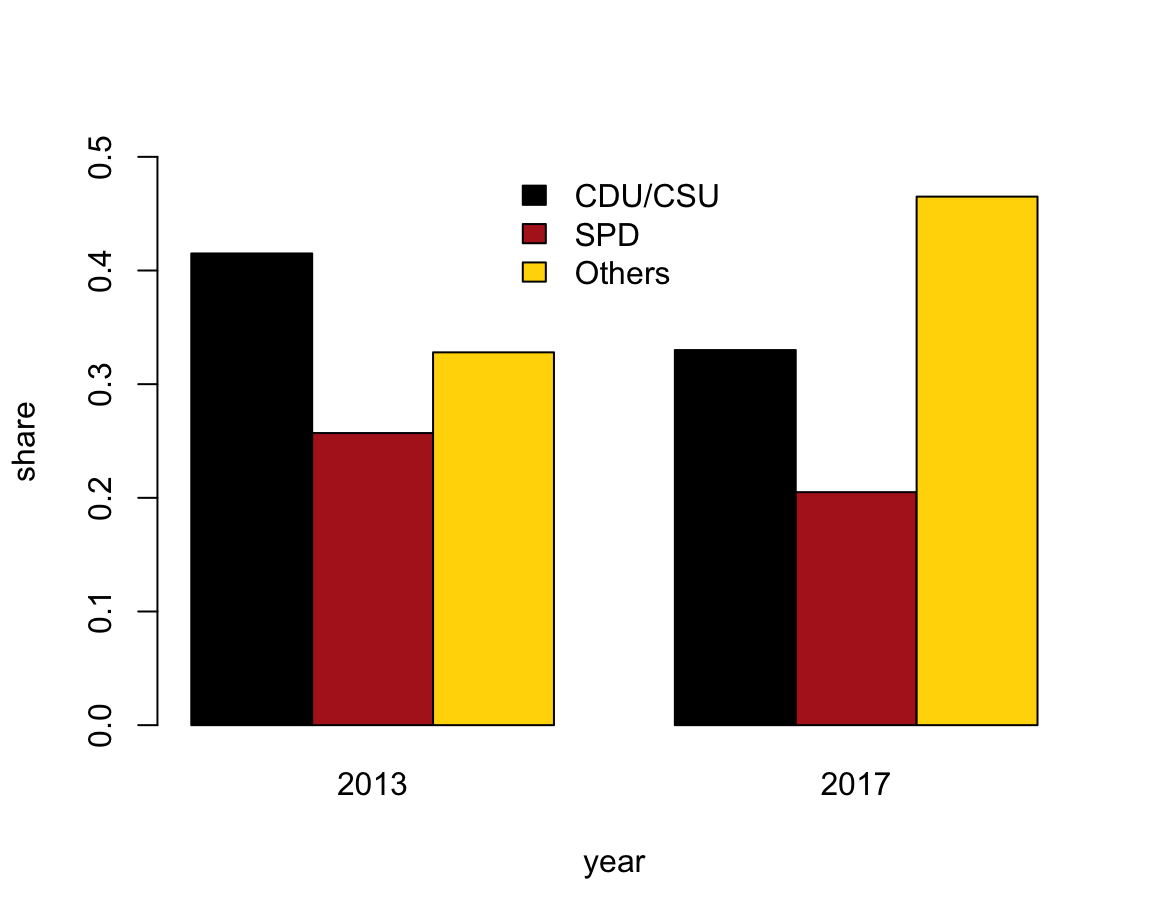### 4.3.4 Box plots

A common situation in most sciences consists in the following scenario:
We want to describe the value(s) of some quantitative variable (i.e., some dependent variable $$y$$) as a function of a categorical variable (i.e., some independent variable $$x$$).

For instance, given the mpg dataset, we could wonder:

• How does a car’s average fuel consumption in the city (i.e., the dependent variable cty) vary as a function of its type (or independent variable class)?

The following Table 4.3 describes this information:

Table 4.3: Average MPG in cty as a function of a car’s class.
class n mean SD
2seater 5 15.40000 0.5477226
compact 47 20.12766 3.3854999
midsize 41 18.75610 1.9465416
minivan 11 15.81818 1.8340219
pickup 33 13.00000 2.0463382
subcompact 35 20.37143 4.6023377
suv 62 13.50000 2.4208791

How can we express such information in a visual format? A standard solution consists in creating a so-called boxplot. Boxplots are also known as box-and-whisker plots (see Wikipedia: Box plot for details) and were promoted by John Tukey in the 1970s (e.g., Tukey, 1977).

In R, we can create boxplots by using the boxplot() function. Its data argument allows specifying a dataset (typically a dataframe or tibble) and its formula allows specifying a dependent variable dv and an independent variable iv as dv ~ iv. In the context of our example, we could call:

boxplot(formula = cty ~ class, data = mpg)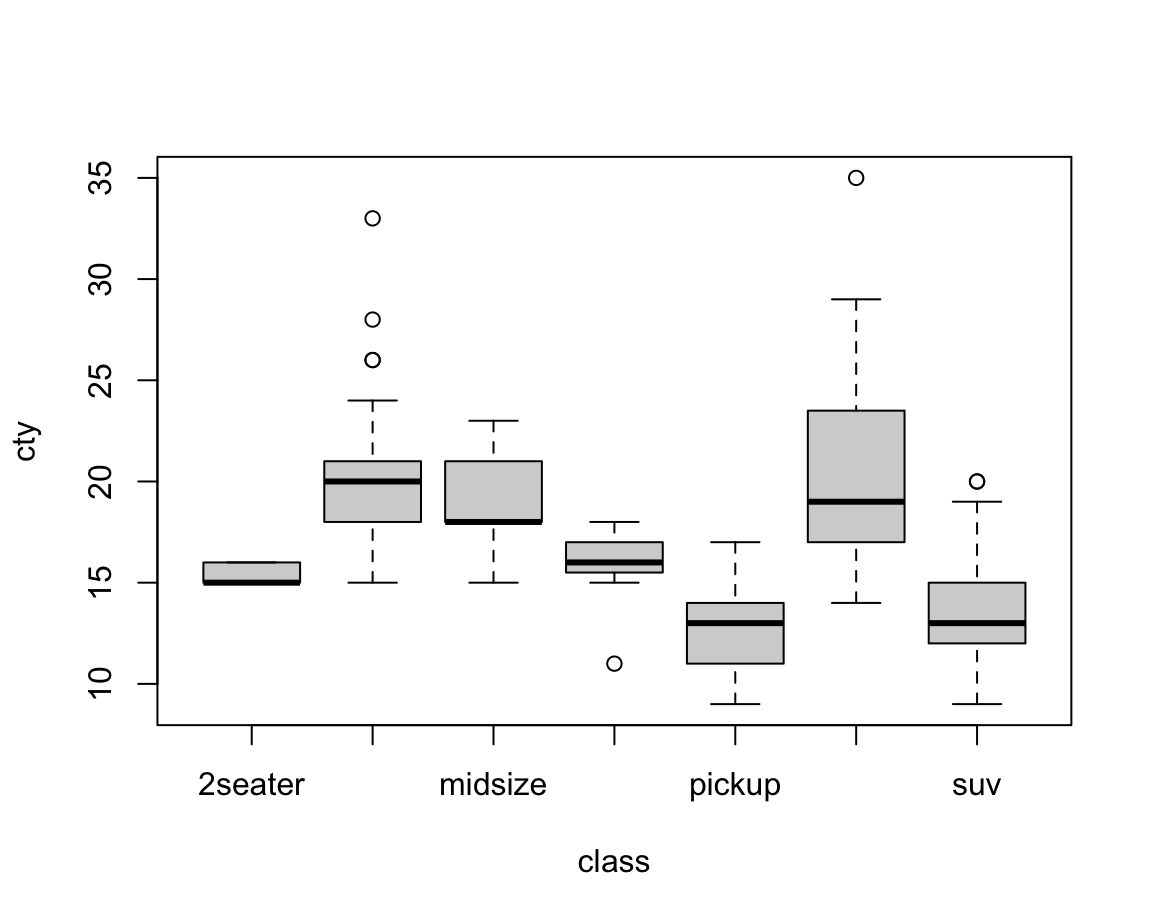Figure 4.2: A bare (base R) boxplot.

A slightly prettier version of the same plot could add some aesthetic features and labels (Figure 4.3):

bp <- boxplot(formula = cty ~ class, data = mpg,
col = car_cols, # using color vector (defined above)
las = 2,        # rotate labels on axes
main = "Box plot on cars' fuel consumption (in city) by class",
xlab = "Vehicle class", ylab = "MPG (in city)")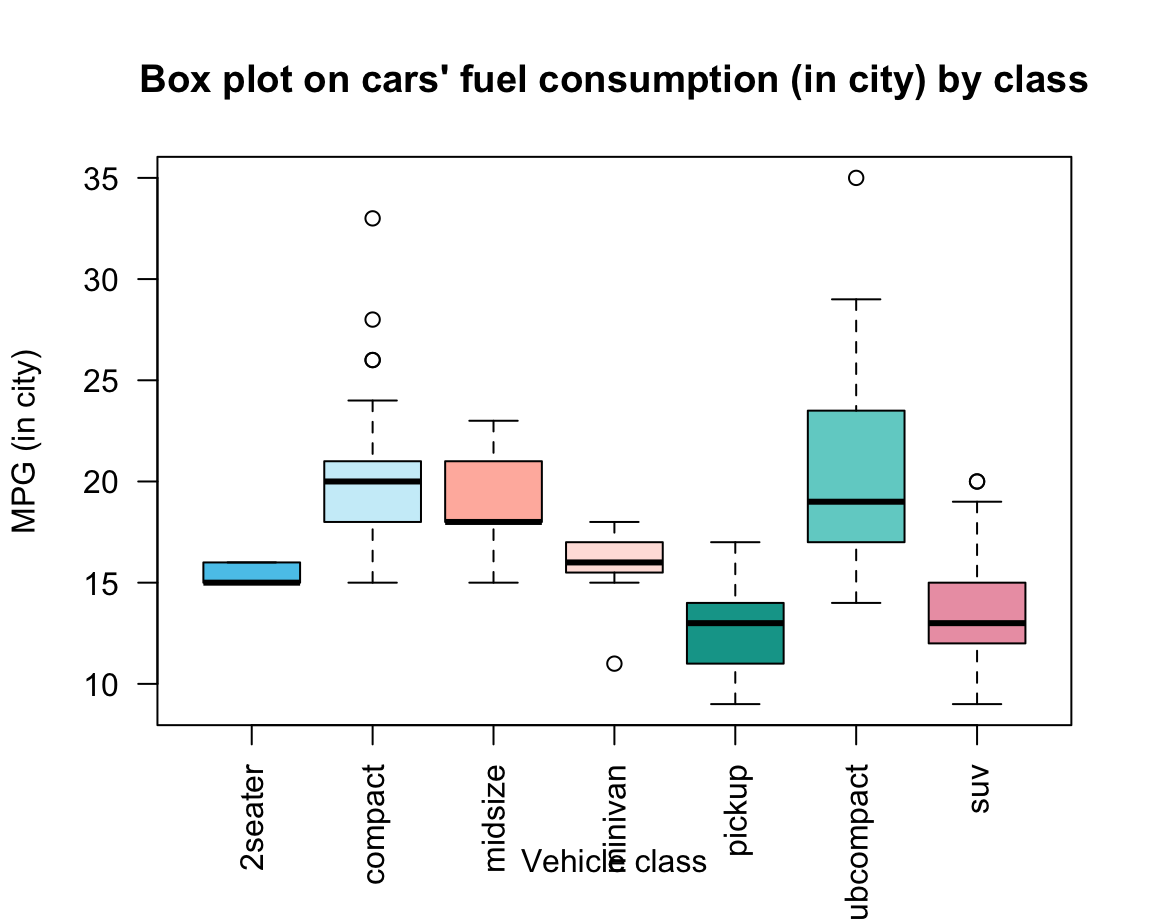Figure 4.3: A boxplot shows non-parametrical information on the mean and variation of each group.

A boxplot provides a standardized, non-parametrical way of displaying the distribution of group-based data based on a four types of information:

1. the fat horizontal line shows the median $$Md$$ (50th percentile of data) of each group;

2. the box for each group shows the interquartile range ($$IQR$$) around the median $$Md$$ in both directions (i.e., the middle 50% of the data):

• below: $$Q1$$ (25th percentile to $$Md$$) vs.
• above: $$Q3$$ ($$Md$$ to 75th percentile);
1. the whiskers (dotted lines) show the ranges to the bottom 25% and the top 25% of the data values, excluding outliers:
• lower whisker: minimum ($$Q1 - 1.5 \cdot IQR$$) to the
• upper whisker: maximum ($$Q3 + 1.5 \cdot IQR$$);
1. the points beyond the whiskers show the outliers of each group.

Overall, box plots provide information on the center, spread/range, and outliers of each group, without making restrictive assumptions about the distribution of values in a group. Note that the width and shape of the boxes and whiskers also provide information on group size and skew. In Figure 4.3, we can infer that

• the group of “2seater” cars is very homogeneous and probably small (given its tiny variations);
• the group of “midsize” cars is more skewed (many small, some larger values);
• the group of “pickup” cars is more symmetrical than the others.

#### Plots as side effects vs. plot outputs

When creating Figure 4.3, we saved the output of the boxplot() function to an object bp. This illustrates that plots can occasionally return outputs that are worth saving as R objects.

To explain this, we first need to distinguish between the values returned by a function and their side effects. In our chapter on basic R concepts and commands, we have learned that functions are R objects that do stuff and typically return an object or value. However, some functions do more than just returning an object: They change variables in some environment (e.g., the assignment <- function), plot graphics (e.g., plot()), load or save files, or access a network connection. Such additional operations are collectively called the side effects of a function.

Plots typically change something in our interface (by printing a plot or some part of a plot onto a canvas, e.g., in the Plots window), but this has no lasting effect on our code. Unless a plot returns something that we save into an R object it leaves no trace in our current environment — the plot is a mere side effects of a function call. Consider, for instance, our simple example (from above) that created our first plot:

x <- -10:10
y <- x^2
z <- plot(x, y)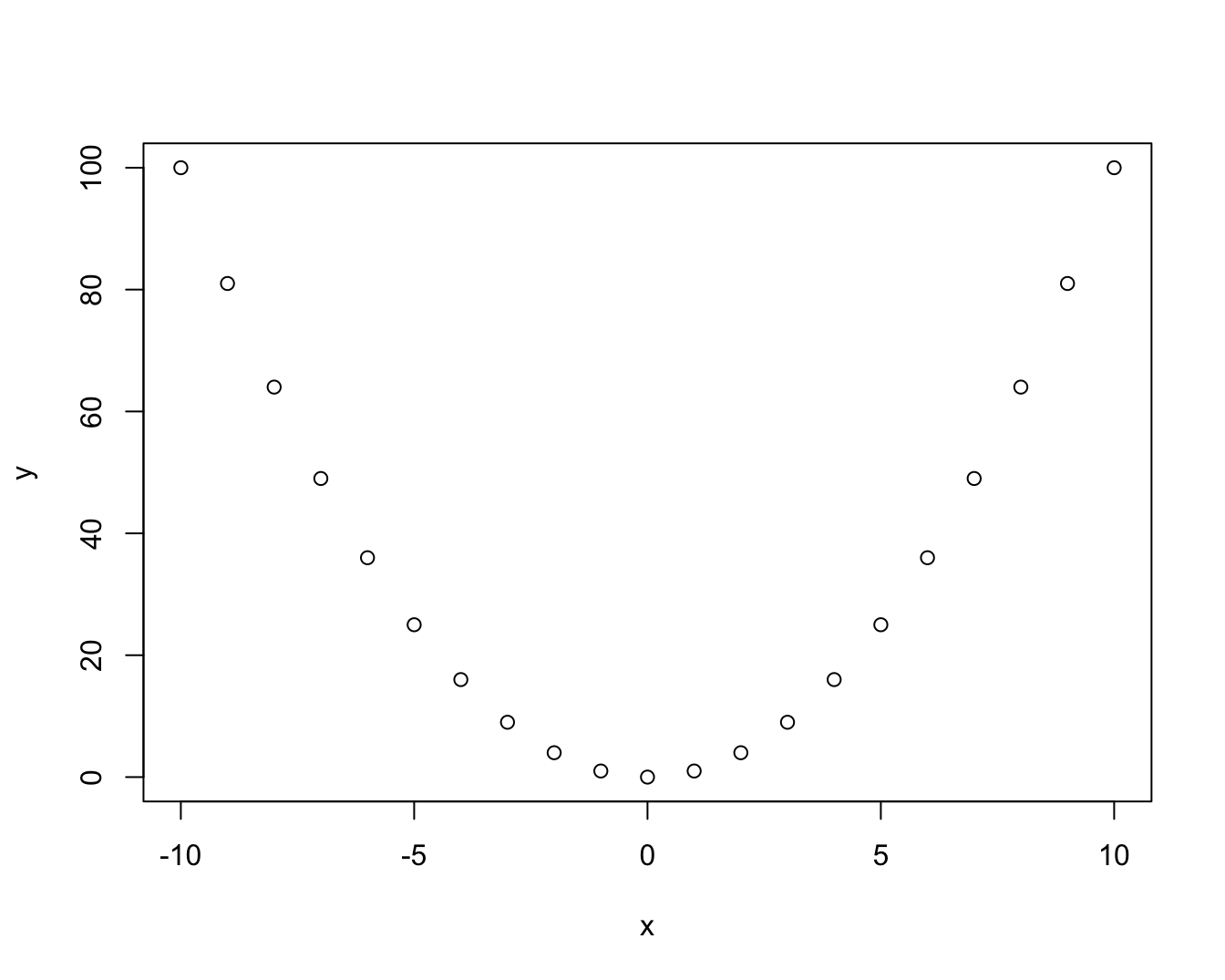z
#> NULL

This plot is printed to some electronic canvas (a so-called R plotting device). Further using or changing this plot as an R object would require either (a) that the plot() function returns an object which we can further manipulate, or (b) that we obtain additional functions that manipulate the same canvas (or plotting device). As we will see shortly, base R typically opts for option (b): Plots are created as side effects of functions and other functions can add more side effects to the same plot. This allows incrementally creating to a plot, and then changing it as long as we can access the same canvas (or device).

We could ask: What object does the plot() function return? Well, we just tested this by assigning its output to an object z and asking R to evaluate z. The answer of NULL here is R’s way of saying: This object contains nothing (but has been defined and is not NA). In the present context, it means that plot() did not return anything we can further work with in our current R environment.

By contrast, the boxplot() function does return an R object we can work with. In the documentation ?boxplot we can read that it returns a value as a list with various components (called stats, n, conf, etc.). Thus, our reason for assigning its output to an object bp was to later access these components (e.g., by the $ notation for accessing list elements): bp$n      # size of groups
#>   5 47 41 11 33 35 62
bp$names # names of groups #>  "2seater" "compact" "midsize" "minivan" "pickup" "subcompact" "suv" bp$stats  # summary of stats
#>      [,1] [,2] [,3] [,4] [,5] [,6] [,7]
#> [1,]   15   15   15 15.0    9 14.0    9
#> [2,]   15   18   18 15.5   11 17.0   12
#> [3,]   15   20   18 16.0   13 19.0   13
#> [4,]   16   21   21 17.0   14 23.5   15
#> [5,]   16   24   23 18.0   17 29.0   19
#> attr(,"class")
#>   2seater
#> "integer"
bp$conf # confidence intervals (of notch) #> [,1] [,2] [,3] [,4] [,5] [,6] [,7] #> [1,] 14.2934 19.3086 17.25974 15.28542 12.17487 17.26405 12.39802 #> [2,] 15.7066 20.6914 18.74026 16.71458 13.82513 20.73595 13.60198 bp$out    # outliers (beyond notch)
#>  26 28 26 33 11 35 20 20
bp\$group  # group to which outlier belongs
#>  2 2 2 2 4 6 7 7

All this may sound a bit complicated, but illustrates a really powerful aspect of R: Functions can do stuff on the side (e.g., create a visualizations on some plotting device), but still return complicated objects that later allow us accessing particular details of a result. As functions get more complex (e.g., when performing some statistical analysis), this is R’s way of offering a variety of results all at once.

### 4.3.5 Curves and lines

Before turning to more complex plots, we consider two more functions that are often needed when creating plots in base R. The function

• curve() computes and plots the values of a function — specified by expr — for a given range of values.

• abline() computes and plots the values of a linear function (specified by their intercept a and slope b, or a vertical value v, or a horizontal value h).

Both functions seem quite similar, yet allow illustrating an inconsistency in the base R graphic system: The curve() function has an argument add that is FALSE by default, which means that a new plot is created whenever calling the function. To add a curve to an existing plot, we need to specify add = TRUE. By contrast, the abline() function assumes that a plot exists (i.e., some variant of plot() has been evaluated before calling abline()). In fact, abline() actually issues an error that plot.new has not been called yet when it is called without an existing plot.

Such quirks and inconsistencies are inevitable when using a graphics system that has evolved for over 30 years. Once we successfully navigate them, the system is quite powerful for creating a variety of curves and lines:

# Creating a curve plot:
curve(expr = x^2, from = -10, to = +10,
ylab = "y-value", main = "Curvy plots")

# Adding curves to an existing plot:
curve(expr = x^3/5 + 50, col = "red", add = TRUE)
curve(expr = 20 * sin(x) + 50, col = "gold", add = TRUE)

# Adding lines to an existing plot:
curve(expr = -4 * x + 40, col = "green", add = TRUE)  # curve can be a line
# vs.
abline(a = 50, b = -4, col = "blue")   # a linear function
abline(v =  0, col = "grey", lty = 2)  # a vertical (dashed) line
abline(h = 50, col = "grey", lty = 3)  # a horizontal (dotted) line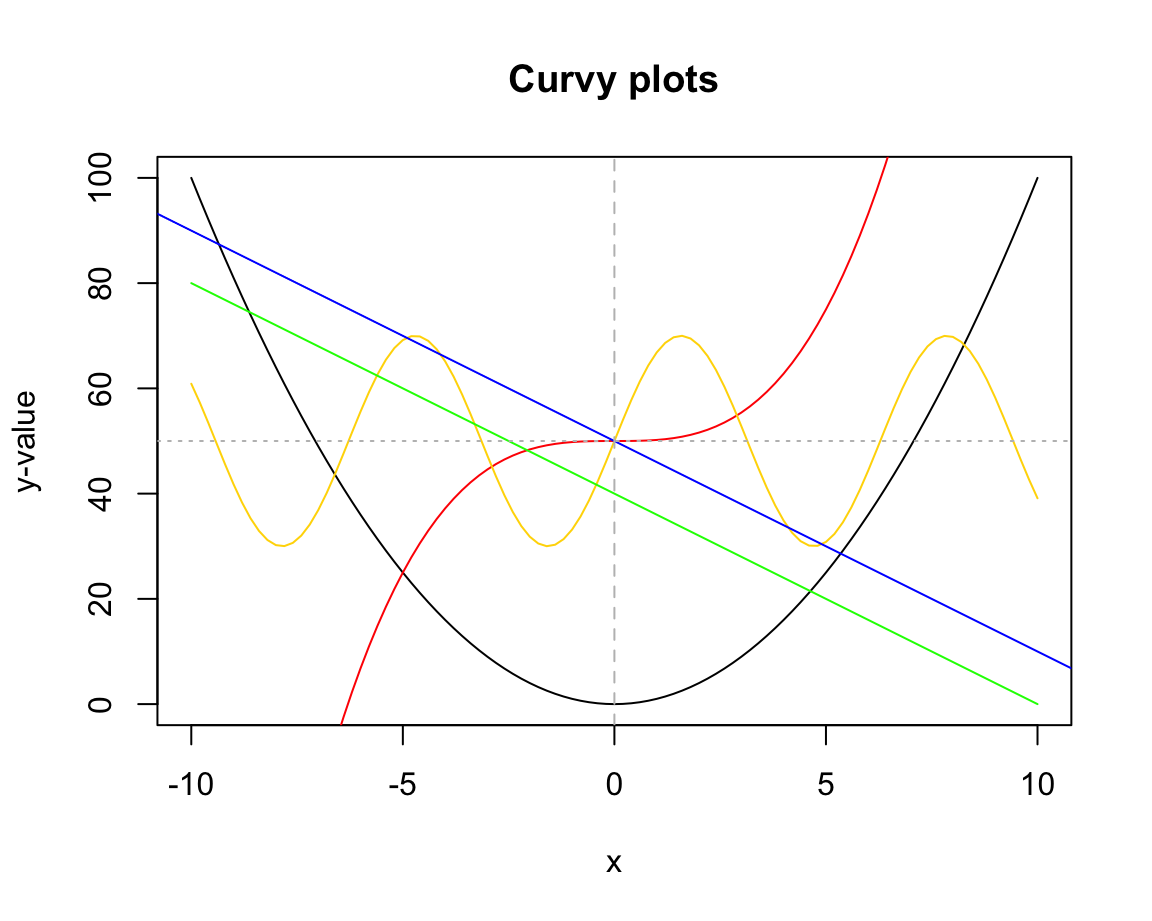Figure 4.4: Comparing the curve() and abline() functions of base R.

Figure 4.4 illustrates another difference between both functions: curve() only plots the specified function over the specified interval (from, to), whereas abline() plots the function for the entire range of x-values.

### 4.3.6 Other plots

There are base R functions for many additional types of visualizations. Many of them are highly specialized and are rarely used. But as they can be useful in particular purposes, it is good to be aware of their existence. Here are some examples:

• Stripcharts create a 1-D scatter plot — perhaps the most basic plot available:
# Create a vector of random values:
set.seed(123)  # reproducible randomness
v <- round(rnorm(n = 50, mean = 10, sd = 2), 0)  # rounded to nearest integer

stripchart(v)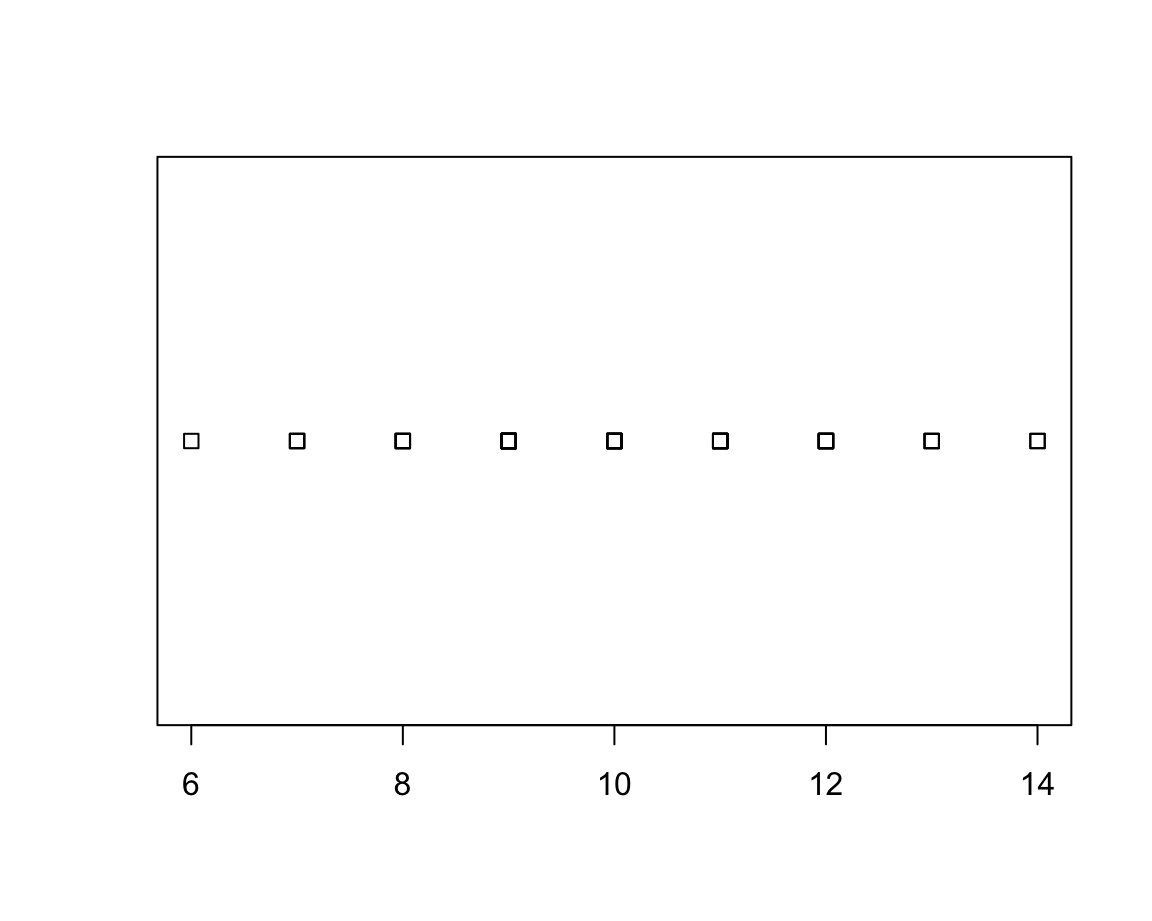The default method = "overplot" is only useful if there are no repeated values. If there are, the method settings "stack" or "jitter" distribute the values that would otherwise be plotted at the same location: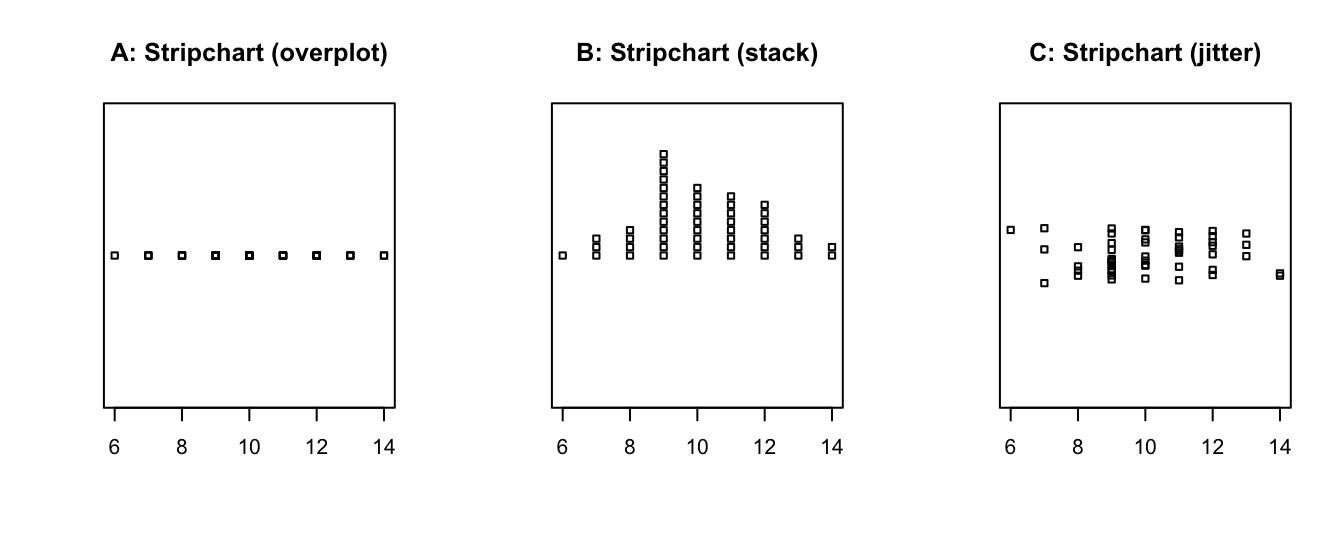• The smoothScatter() function produces density plots, with the option of marking outliers:
N <- 200
x <- rnorm(n = N, mean = 50, sd = 10)
y <- runif(N, min = 0, max = 100)

smoothScatter(x, y, nrpoints = 10, col = "gold", cex = 1, pch = 16)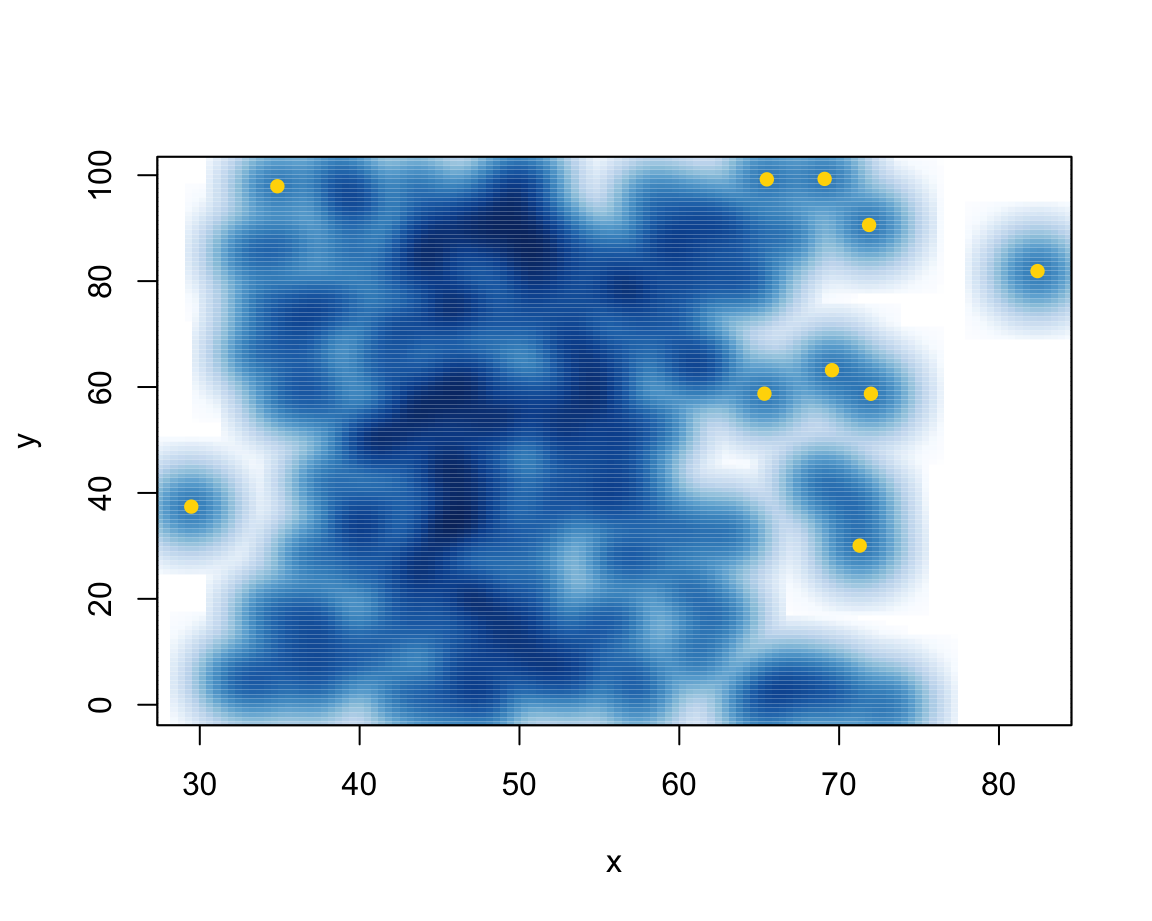• Pie charts rarely make sense and are to be avoided:
c_data <- c(20, 12, 3, 16, 2, 13)
countries <- c("U.S.", "U.K.", "Austria", "Germany", "Switzerland", "France")
pie(c_data, labels = countries, main = "Pie chart of country data")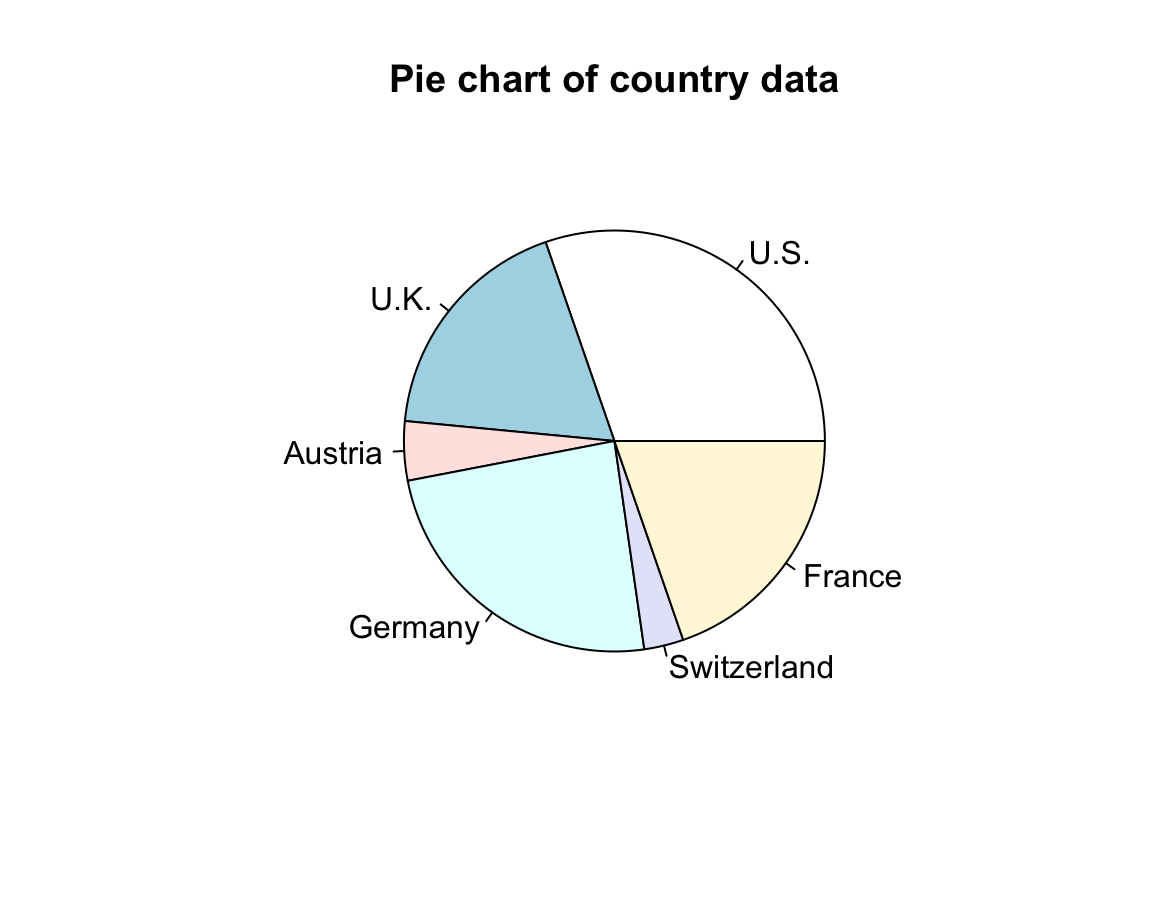• Normal quantile plots:
d <- rnorm(n = 50, mean = 100, sd = 10)
qqnorm(d,
main = "Normal Q-Q plot",
xlab = "Theoretical quantiles",
ylab = "Data quantiles",
asp = 1/10)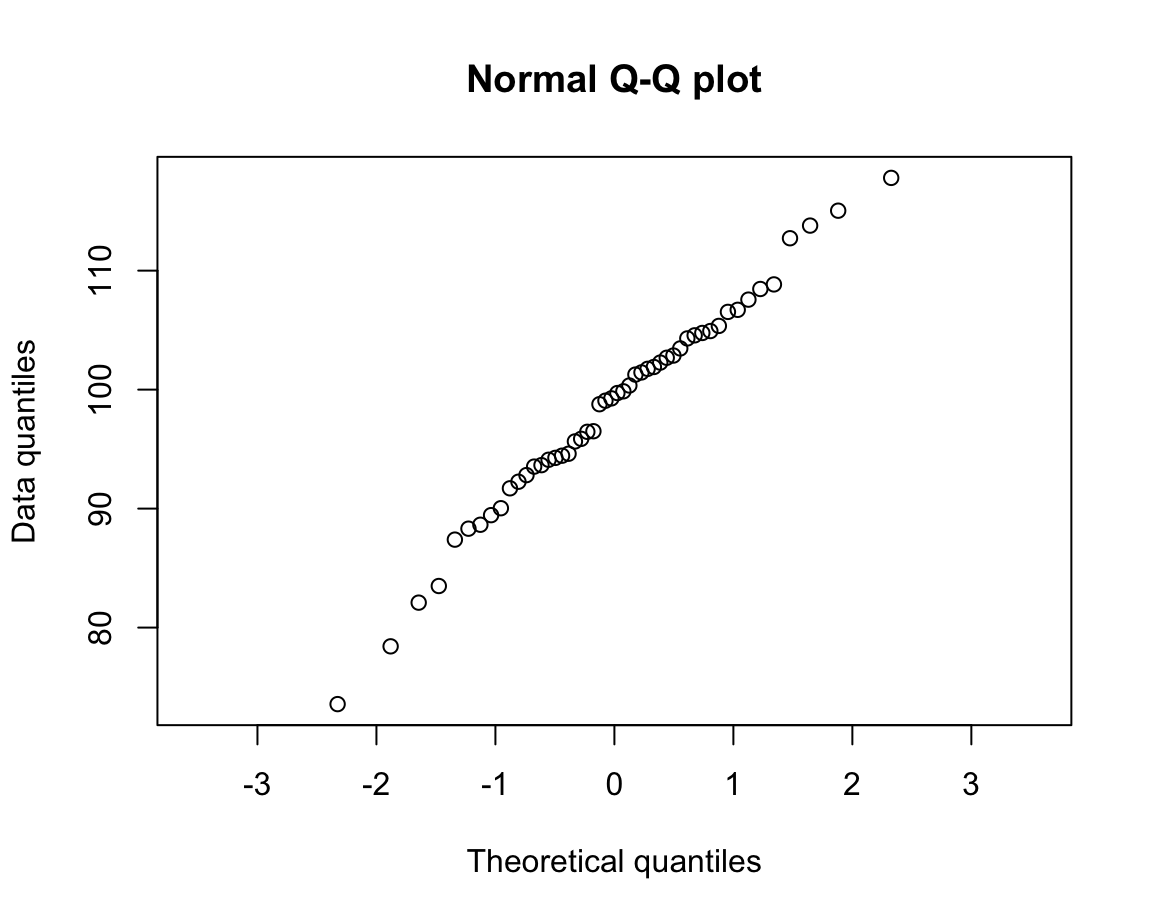qqplot(x, y = qchisq(ppoints(x), df = 3))
abline(a = 0, b = 1, col = "blue", lty = 2)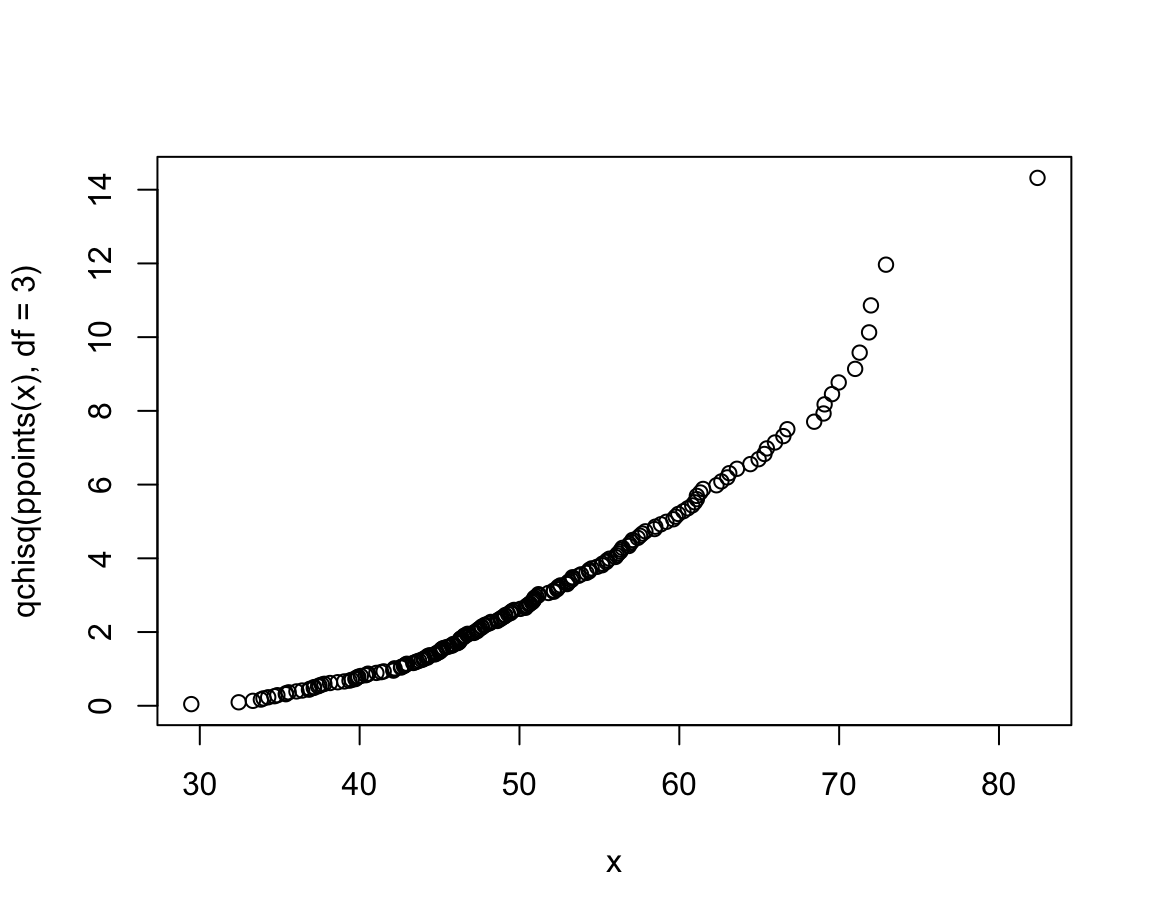Next, we learn how to compose more complex plots as miniature computer programs. As this will give us more control over plot elements, this allows us to create more flexible and powerful visualizations.

### References

Tukey, J. W. (1977). Exploratory data analysis. Addison-Wesley.

1. However, remembering our notion of ecological rationality yields at least two other factors that matter for designing a good visualization.↩︎